#人，一定要靠自己

## 没有人会关心你付出过多少努力，撑得累不累，摔得痛不痛，他们只会看你最后站在什么位置，然后羡慕或者鄙夷

[转载]多维空间点索引算法 — Geohash 和 Google S2
/

## [转载]多维空间点索引算法 — Geohash 和 Google S2## 引子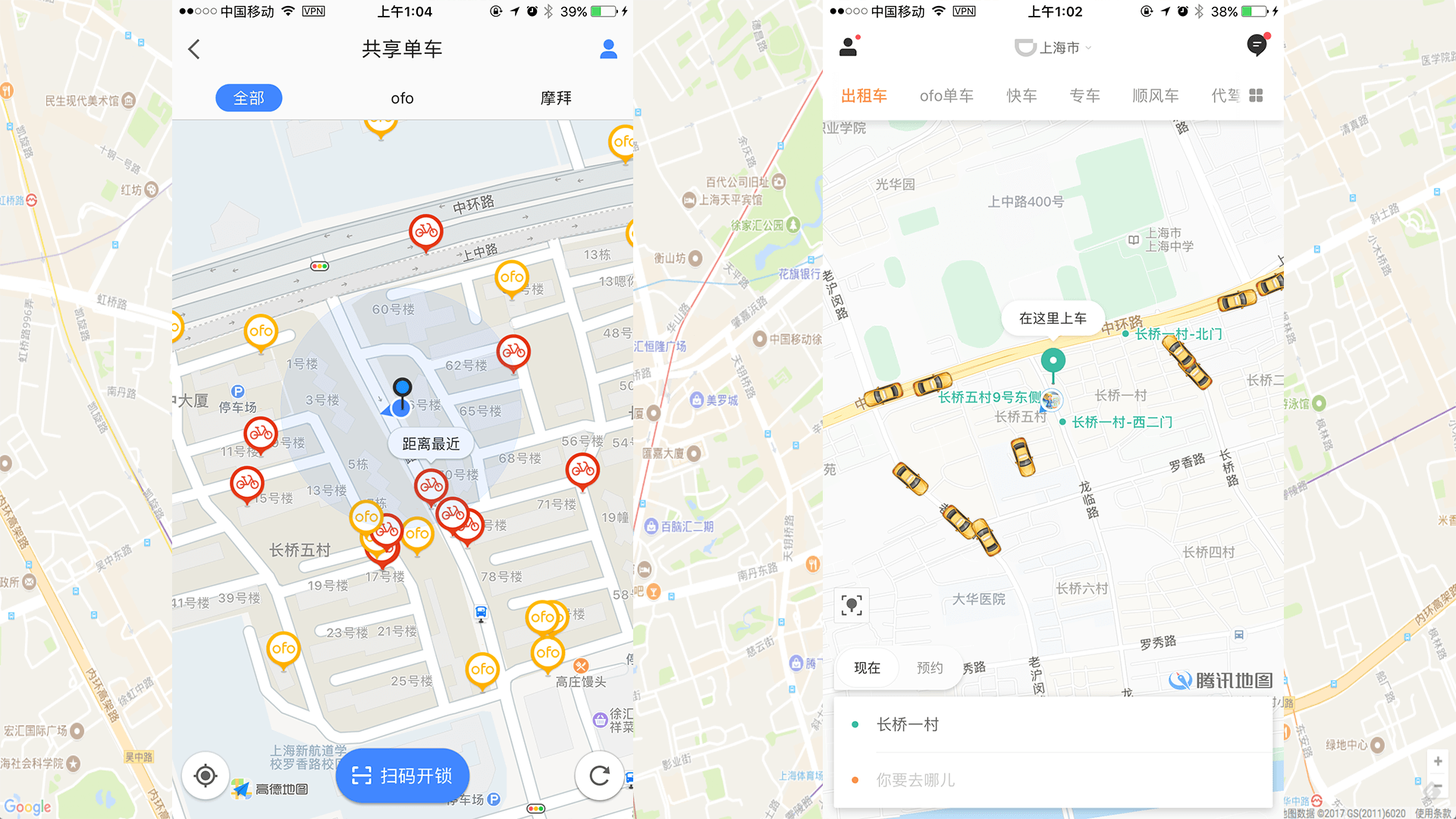app 界面上会显示出自己附近一个范围内可用的出租车或者共享单车。假设地图上会显示以自己为圆心，5公里为半径，这个范围内的车。如何实现呢？最直观的想法就是去数据库里面查表，计算并查询车距离用户小于等于5公里的，筛选出来，把数据返回给客户端。

## 一. GeoHash 算法

### 1. Geohash 算法简介

Geohash 是一种地理编码，由 Gustavo Niemeyer 发明的。它是一种分级的数据结构，把空间划分为网格。Geohash 属于空间填充曲线中的 Z 阶曲线（Z-order curve）的实际应用。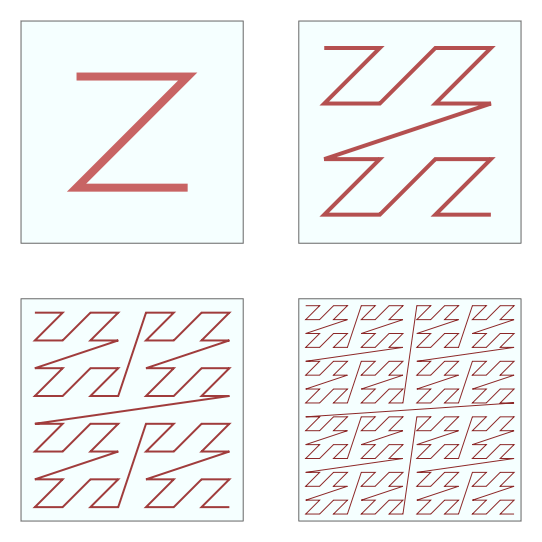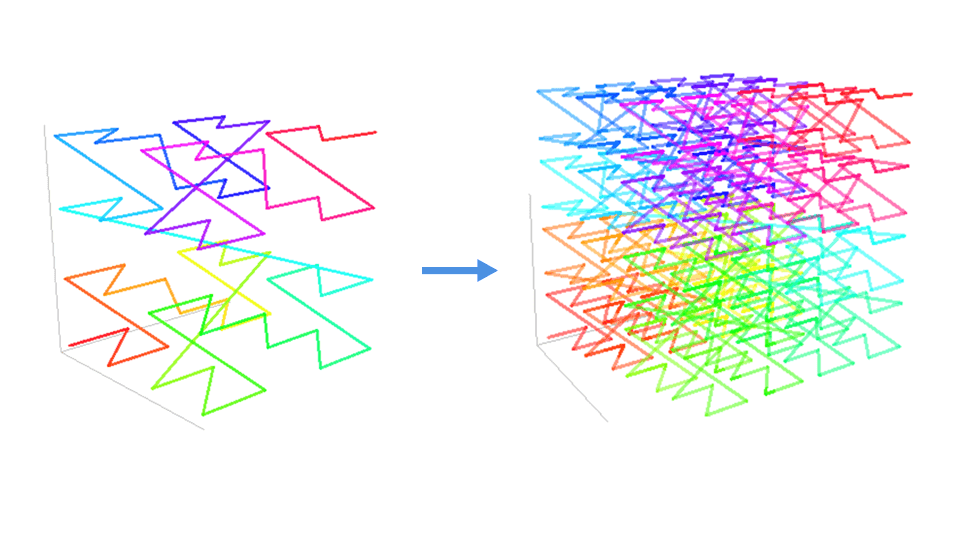Z 阶曲线同样可以扩展到三维空间。只要 Z 形状足够小并且足够密，也能填满整个三维空间。

Geohash 能够提供任意精度的分段级别。一般分级从 1-12 级。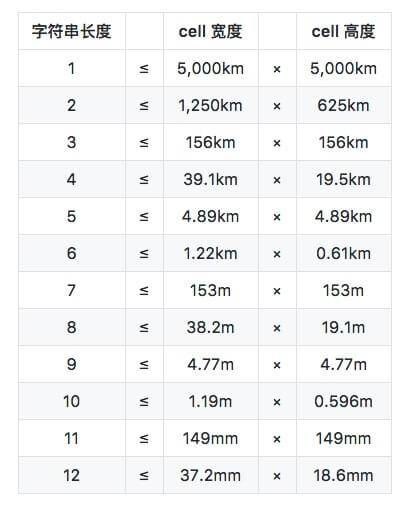Geohash 有一个和 Z 阶曲线相关的性质，那就是一个点附近的地方(但不绝对) hash 字符串总是有公共前缀，并且公共前缀的长度越长，这两个点距离越近。

Geohash 也有几种编码形式，常见的有2种，base 32 和 base 36。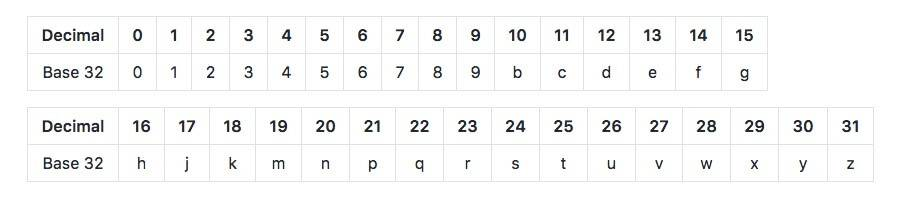base 36 的版本对大小写敏感，用了36个字符，“23456789bBCdDFgGhHjJKlLMnNPqQrRtTVWX”。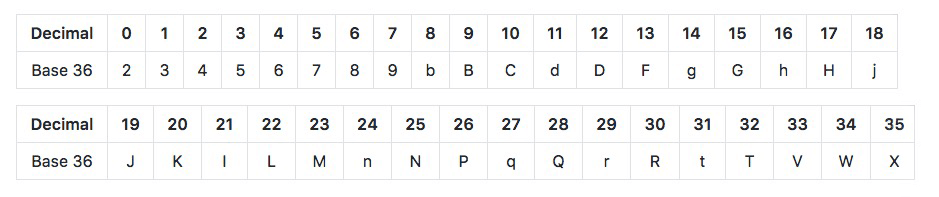### 2. Geohash 实际应用举例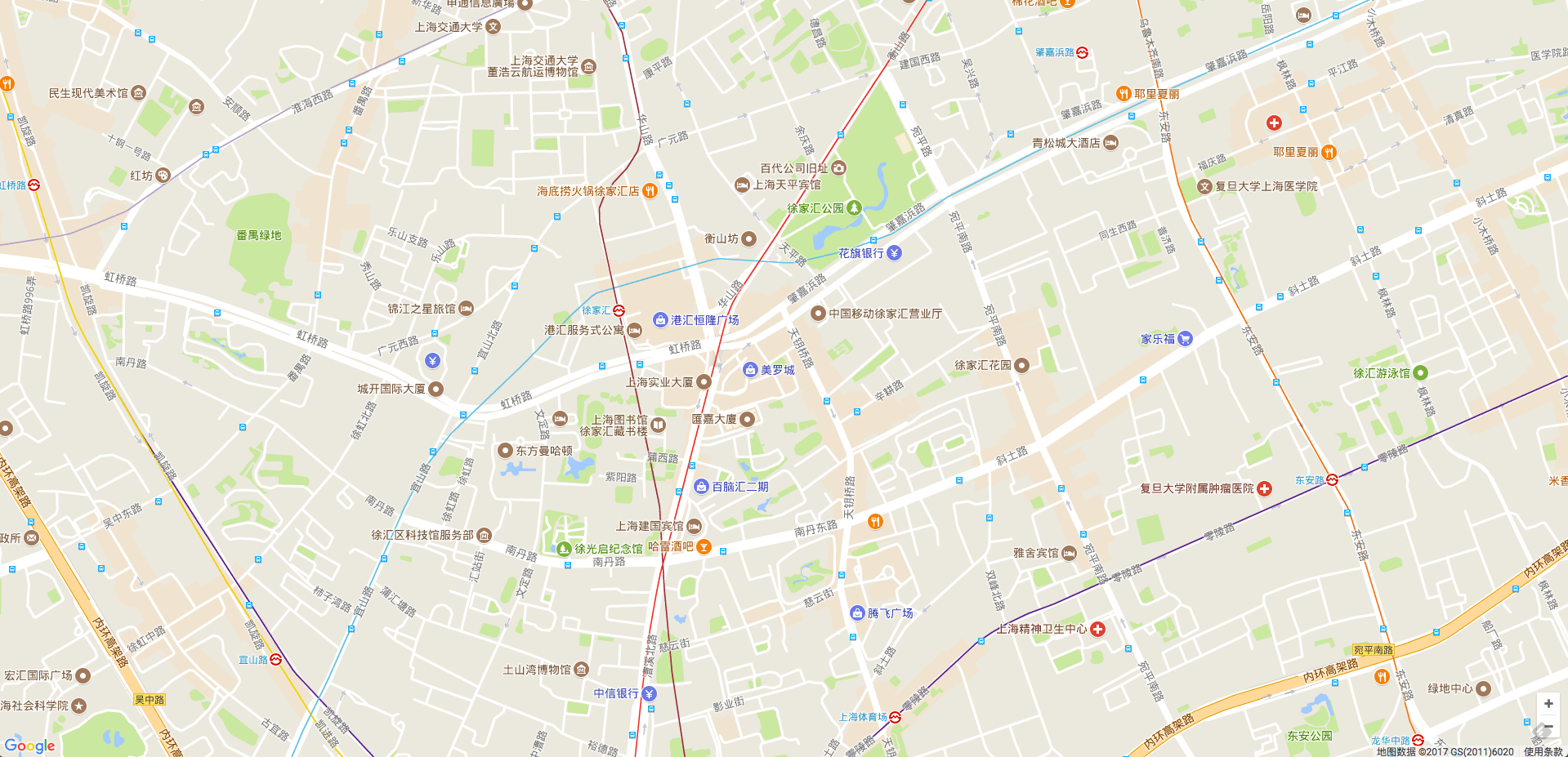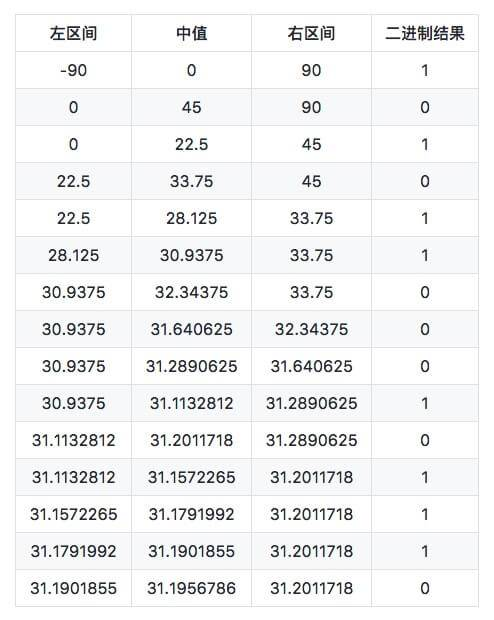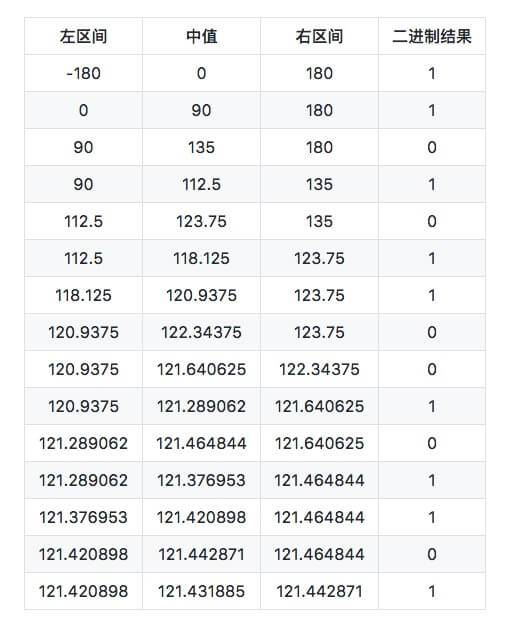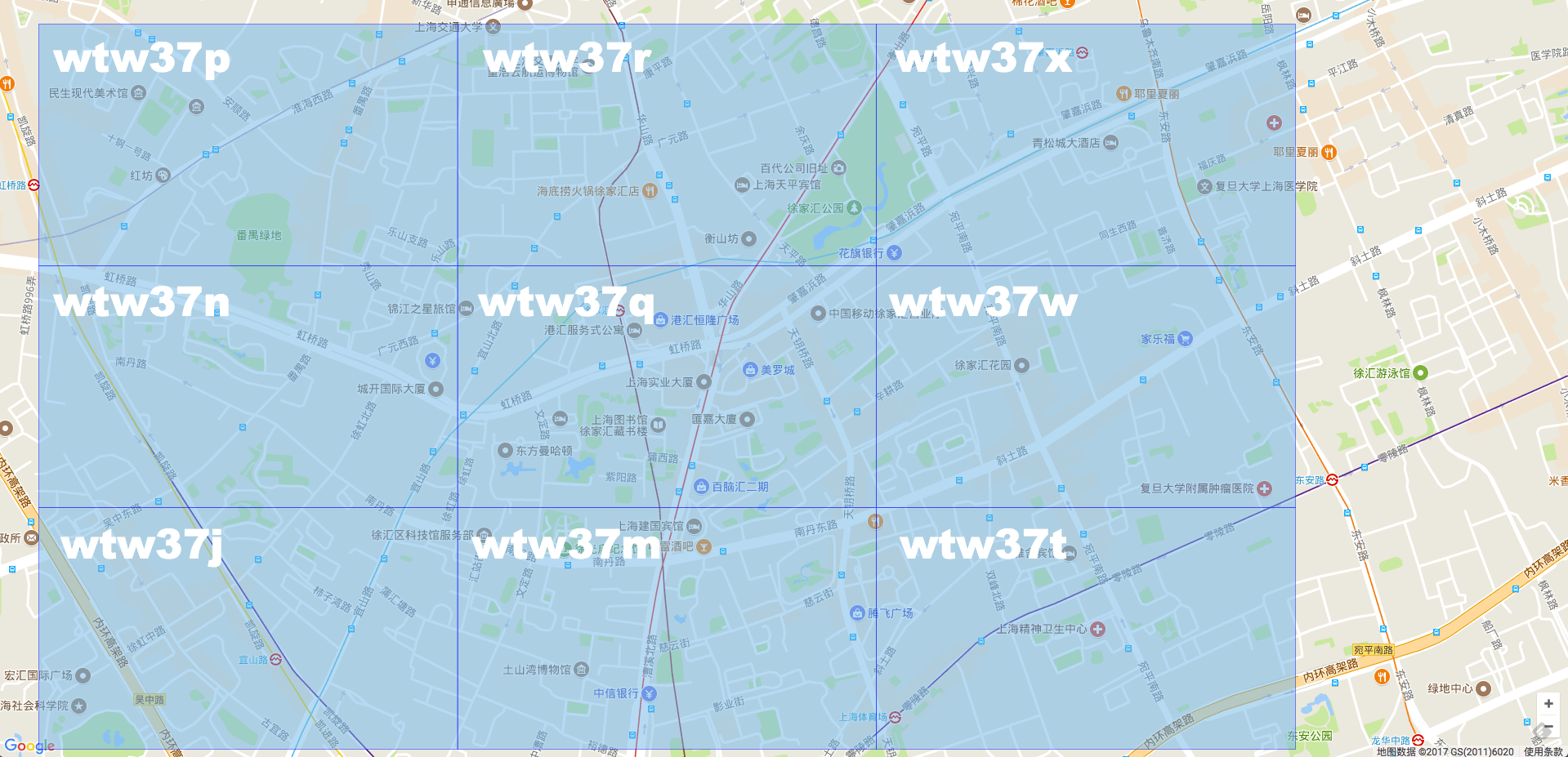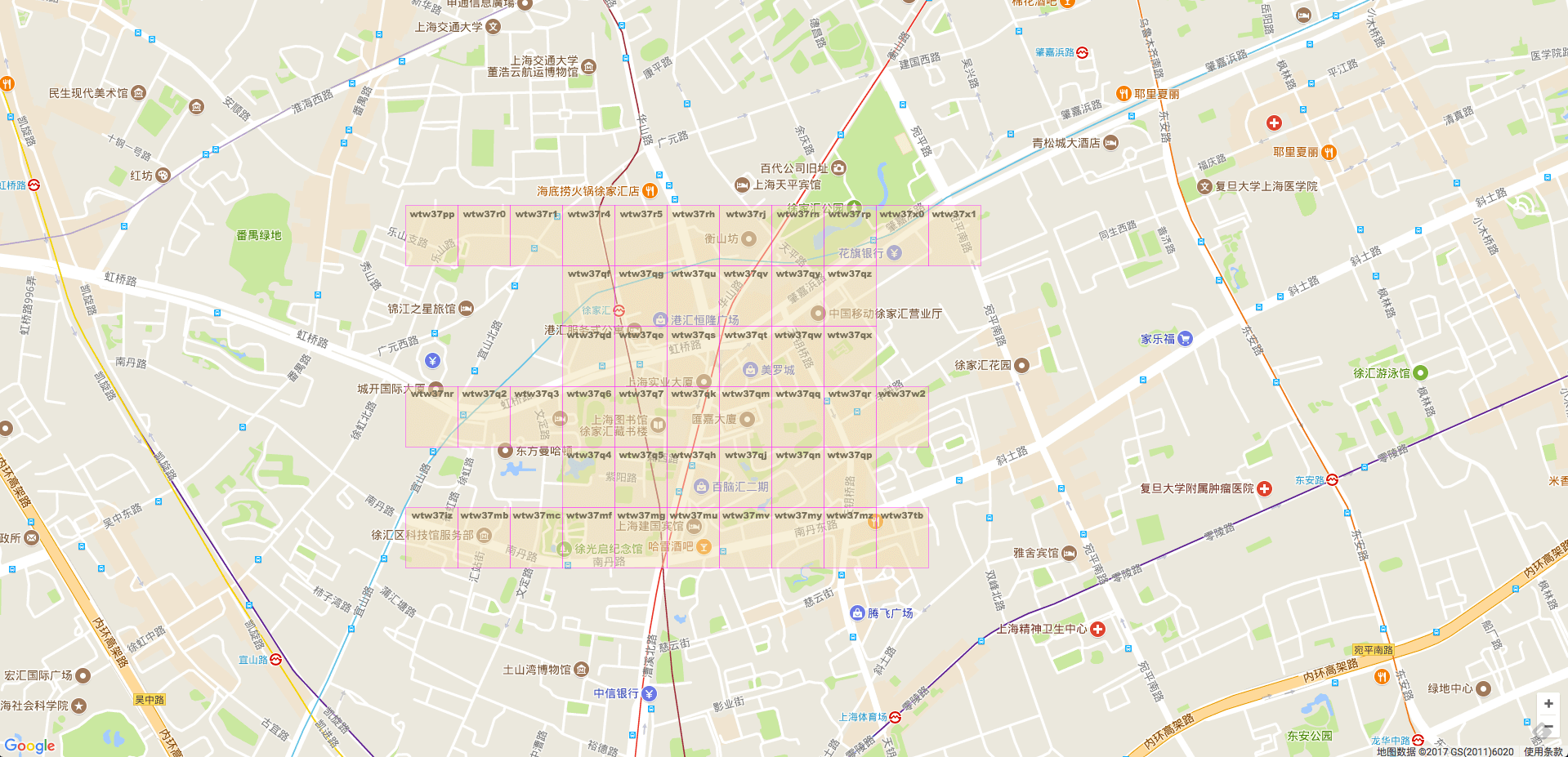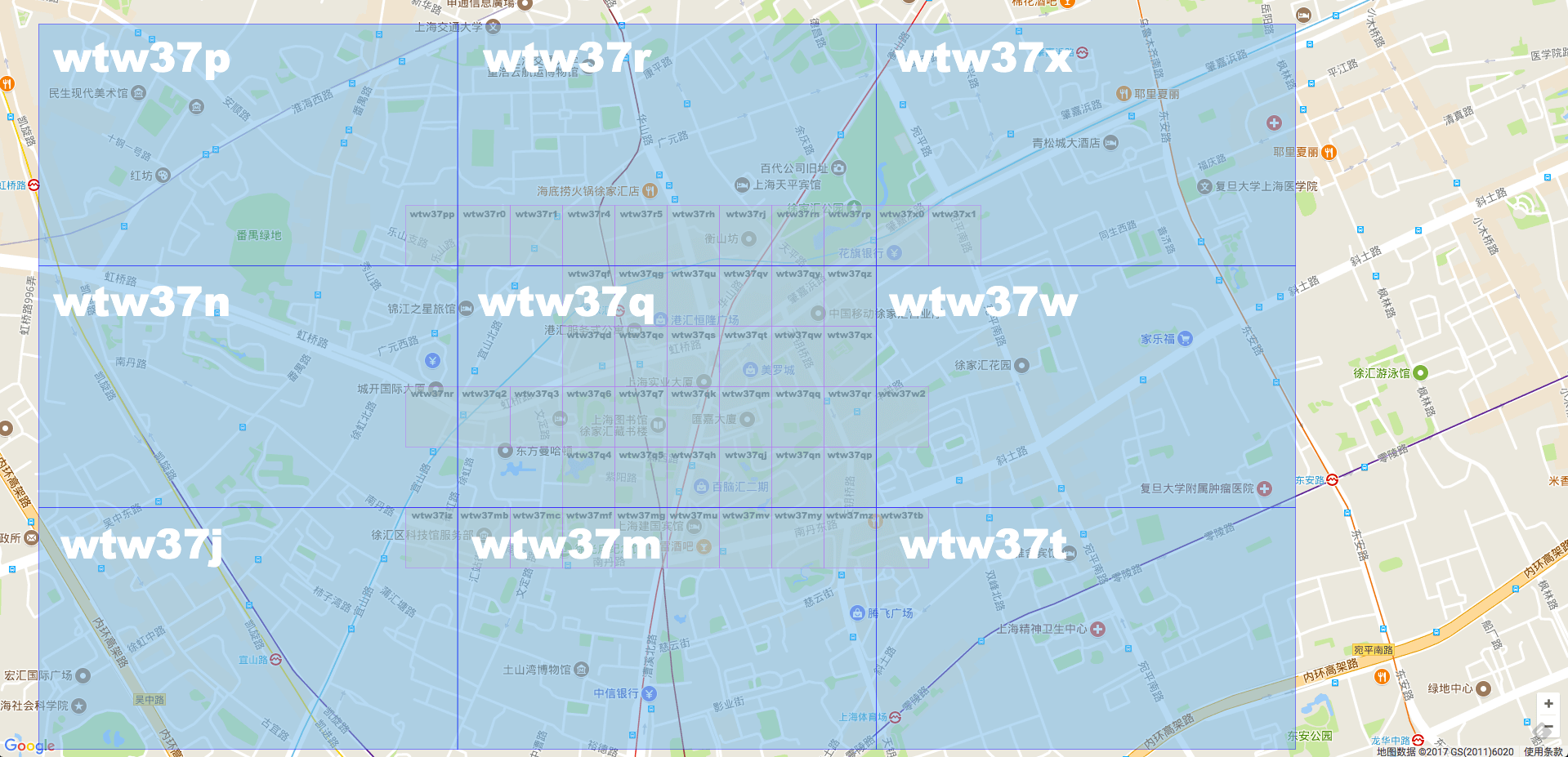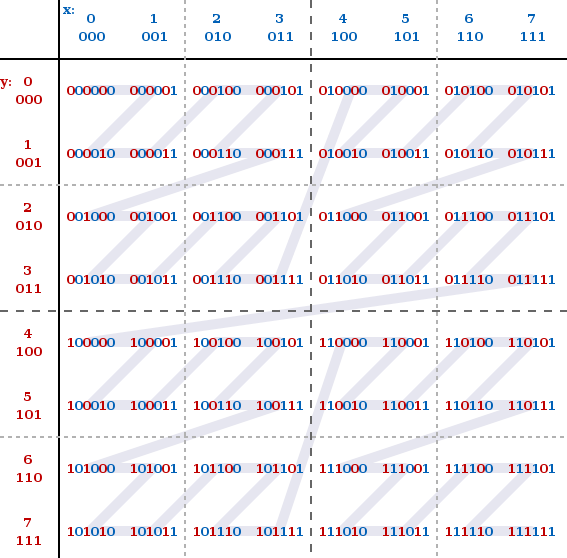x 轴就是纬度，y轴就是经度。经度放偶数位，纬度放奇数位就是这样而来的。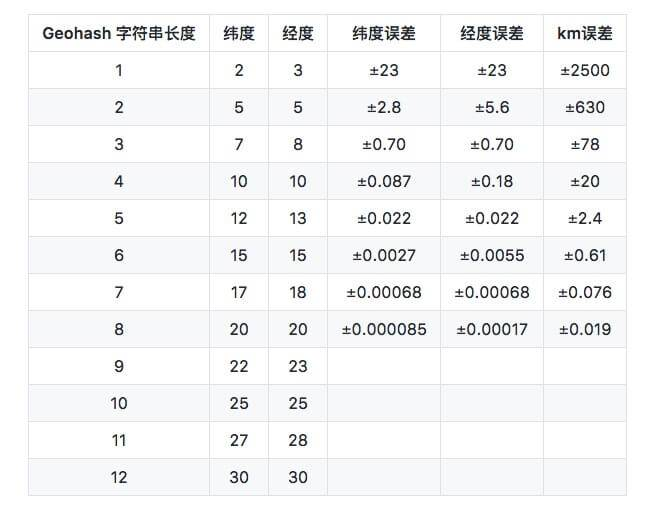### 3. Geohash 具体实现

Go

` package geohash  import (     "bytes" )  const (     BASE32                = "0123456789bcdefghjkmnpqrstuvwxyz"     MAX_LATITUDE  float64 = 90     MIN_LATITUDE  float64 = -90     MAX_LONGITUDE float64 = 180     MIN_LONGITUDE float64 = -180 )  var (     bits   = []int{16, 8, 4, 2, 1}     base32 = []byte(BASE32) )  type Box struct {     MinLat, MaxLat float64 // 纬度     MinLng, MaxLng float64 // 经度 }  func (this *Box) Width() float64 {     return this.MaxLng - this.MinLng }  func (this *Box) Height() float64 {     return this.MaxLat - this.MinLat }  // 输入值：纬度，经度，精度(geohash的长度) // 返回geohash, 以及该点所在的区域 func Encode(latitude, longitude float64, precision int) (string, *Box) {     var geohash bytes.Buffer     var minLat, maxLat float64 = MIN_LATITUDE, MAX_LATITUDE     var minLng, maxLng float64 = MIN_LONGITUDE, MAX_LONGITUDE     var mid float64 = 0      bit, ch, length, isEven := 0, 0, 0, true     for length < precision {         if isEven {             if mid = (minLng + maxLng) / 2; mid < longitude {                 ch |= bits[bit]                 minLng = mid             } else {                 maxLng = mid             }         } else {             if mid = (minLat + maxLat) / 2; mid < latitude {                 ch |= bits[bit]                 minLat = mid             } else {                 maxLat = mid             }         }          isEven = !isEven         if bit < 4 {             bit++         } else {             geohash.WriteByte(base32[ch])             length, bit, ch = length+1, 0, 0         }     }      b := &Box{         MinLat: minLat,         MaxLat: maxLat,         MinLng: minLng,         MaxLng: maxLng,     }      return geohash.String(), b }`

### 4. Geohash 的优缺点

Geohash 的优点很明显，它利用 Z 阶曲线进行编码。而 Z 阶曲线可以将二维或者多维空间里的所有点都转换成一维曲线。在数学上成为分形维。并且 Z 阶曲线还具有局部保序性。

Z 阶曲线通过交织点的坐标值的二进制表示来简单地计算多维度中的点的z值。一旦将数据被加到该排序中，任何一维数据结构，例如二叉搜索树，B树，跳跃表或（具有低有效位被截断）哈希表 都可以用来处理数据。通过 Z 阶曲线所得到的顺序可以等同地被描述为从四叉树的深度优先遍历得到的顺序。

Geohash 的缺点之一也来自 Z 阶曲线。

Z 阶曲线有一个比较严重的问题，虽然有局部保序性，但是它也有突变性。在每个 Z 字母的拐角，都有可能出现顺序的突变。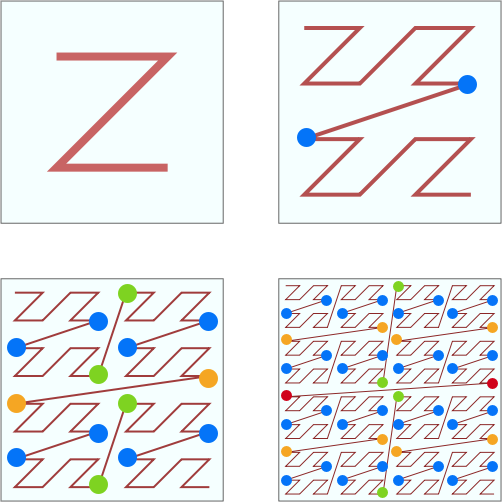Geohash 的另外一个缺点是，如果选择不好合适的网格大小，判断邻近点可能会比较麻烦。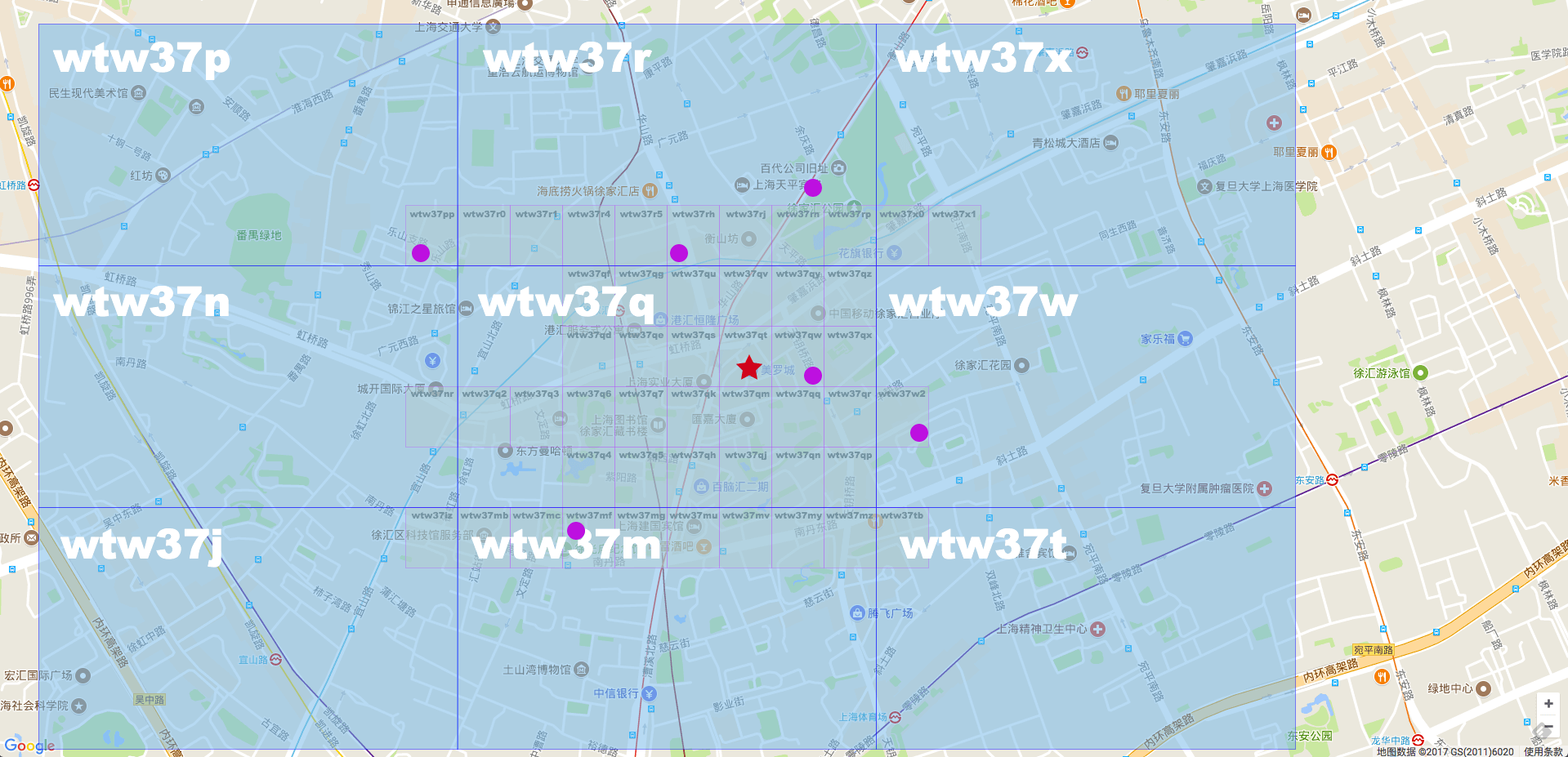## 二. 空间填充曲线 和 分形

### 1. 空间填充曲线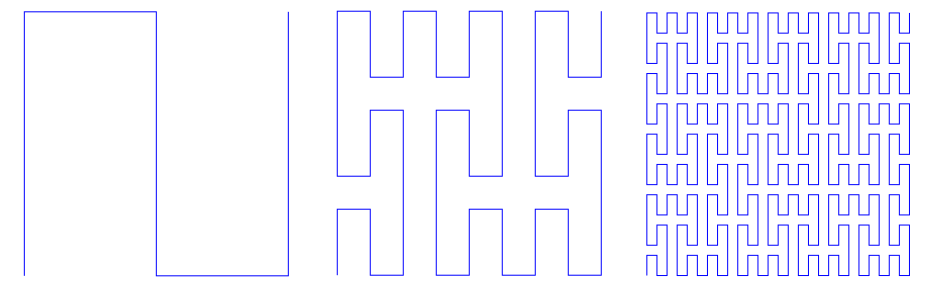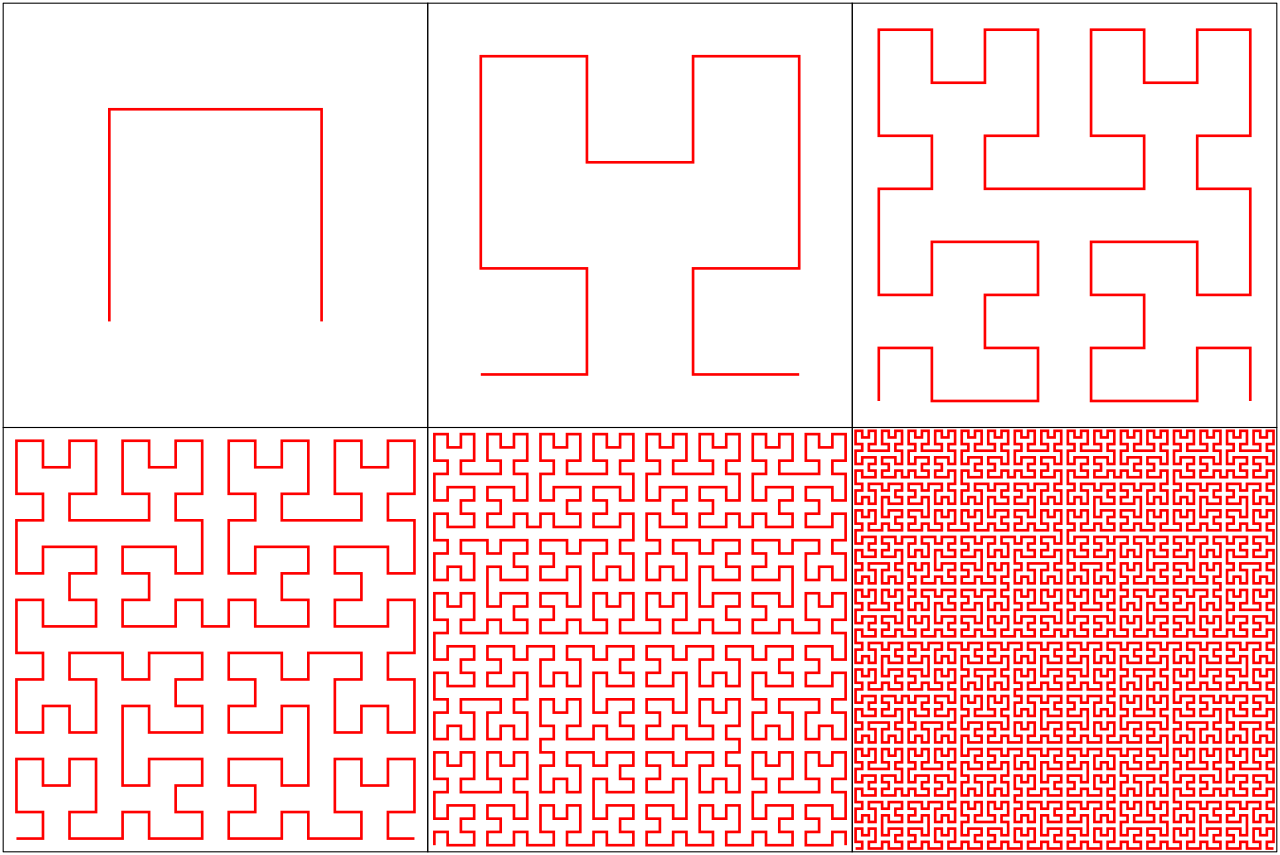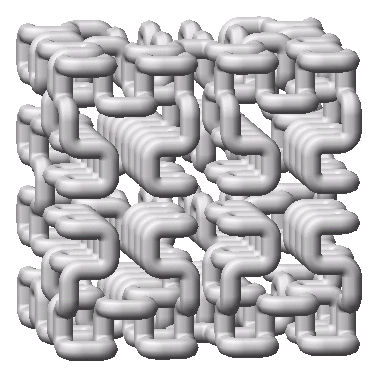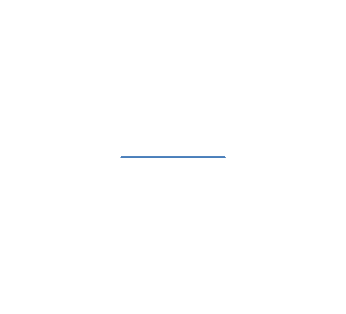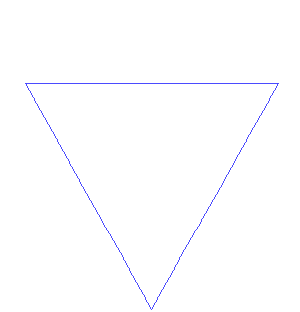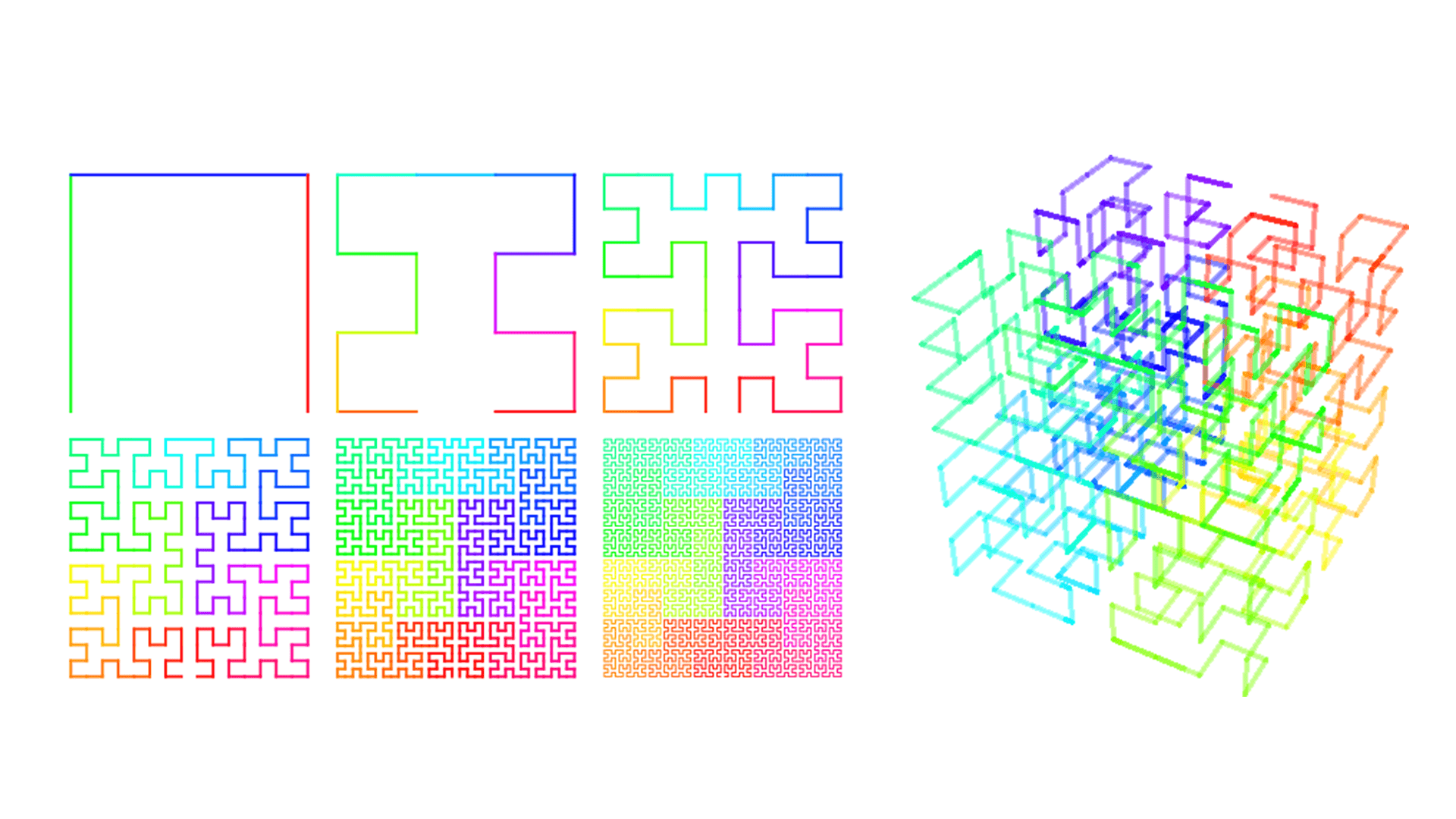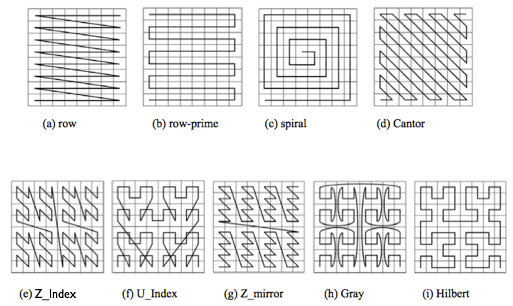## 三. Hilbert Curve 希尔伯特曲线

### 1. 希尔伯特曲线的定义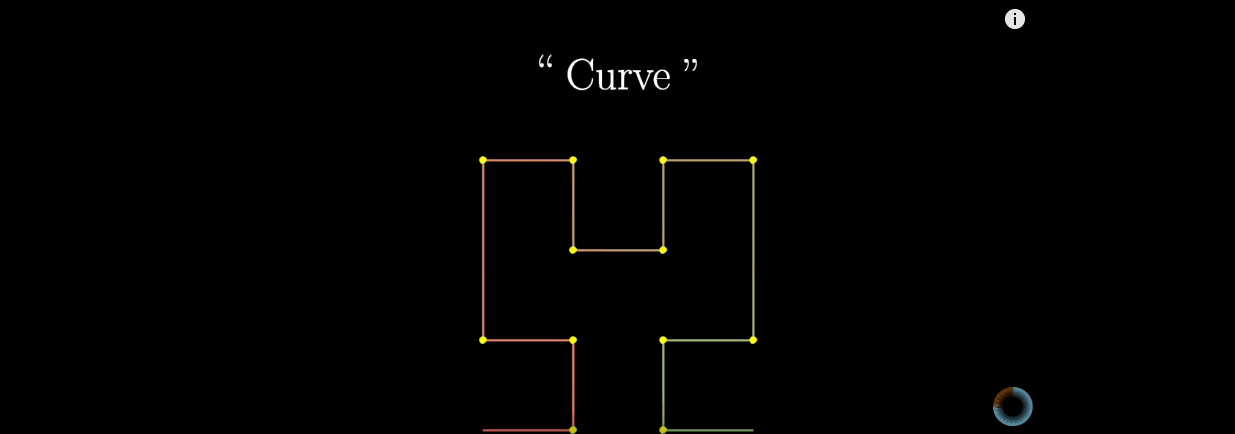### 2. 希尔伯特曲线的构造方法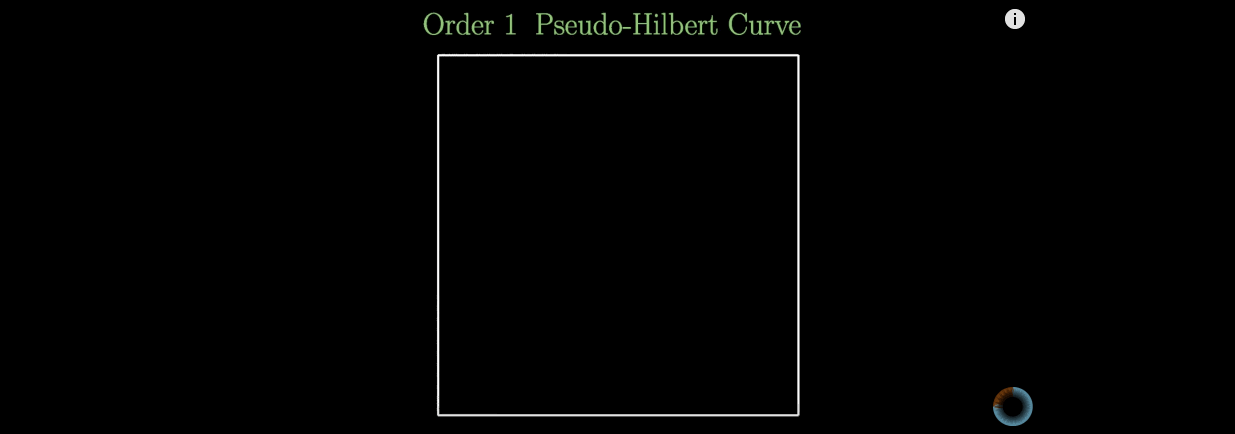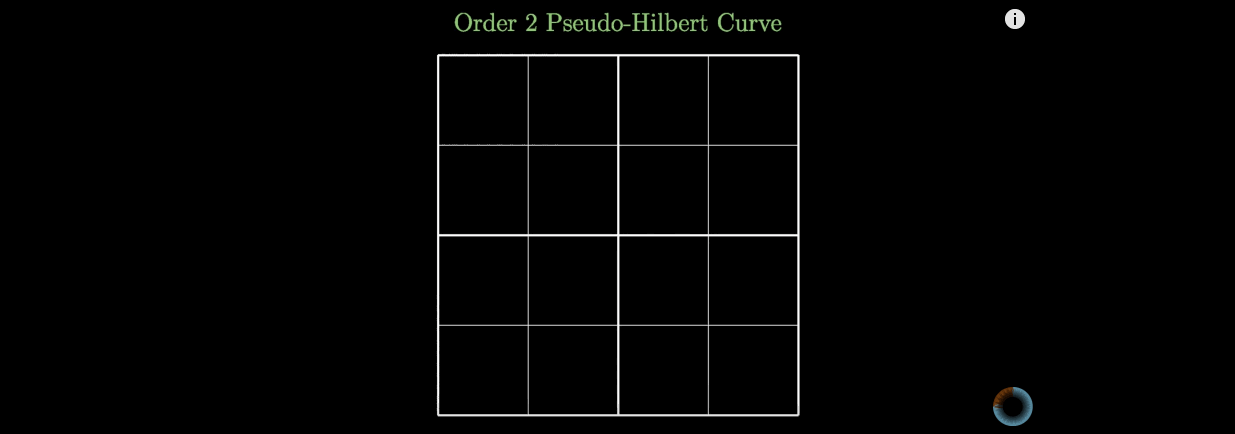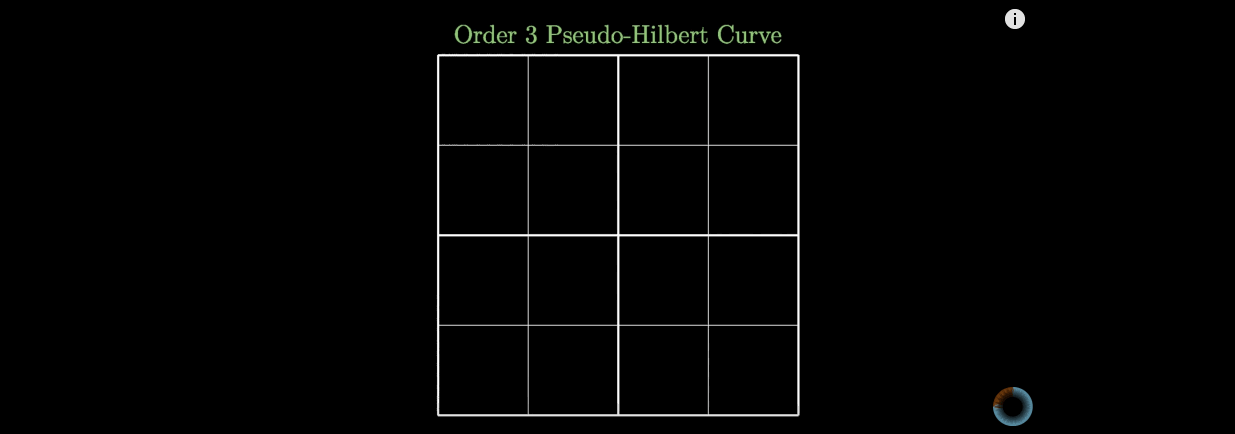n阶的希尔伯特曲线的生成方法也是递归的，先生成n-1阶的希尔伯特曲线，然后把4个n-1阶的希尔伯特曲线首尾相连。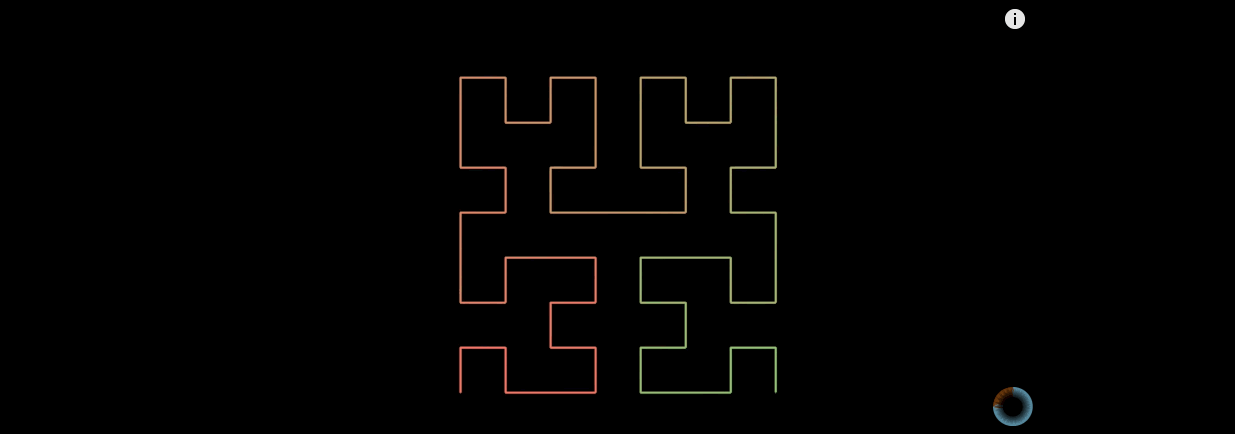### 3. 为何要选希尔伯特曲线

#### (1) 降维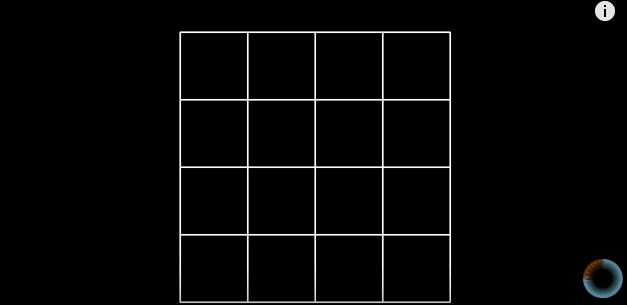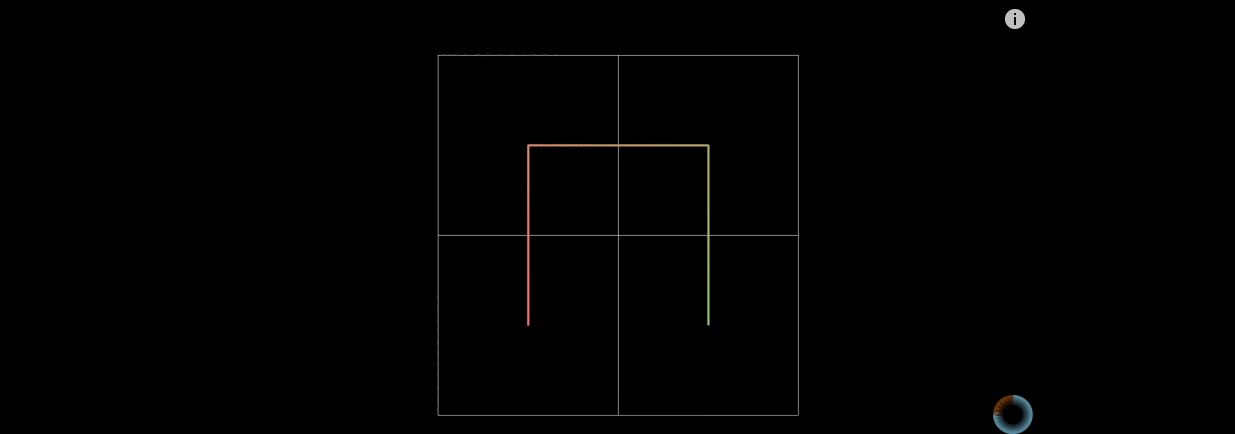#### (2) 稳定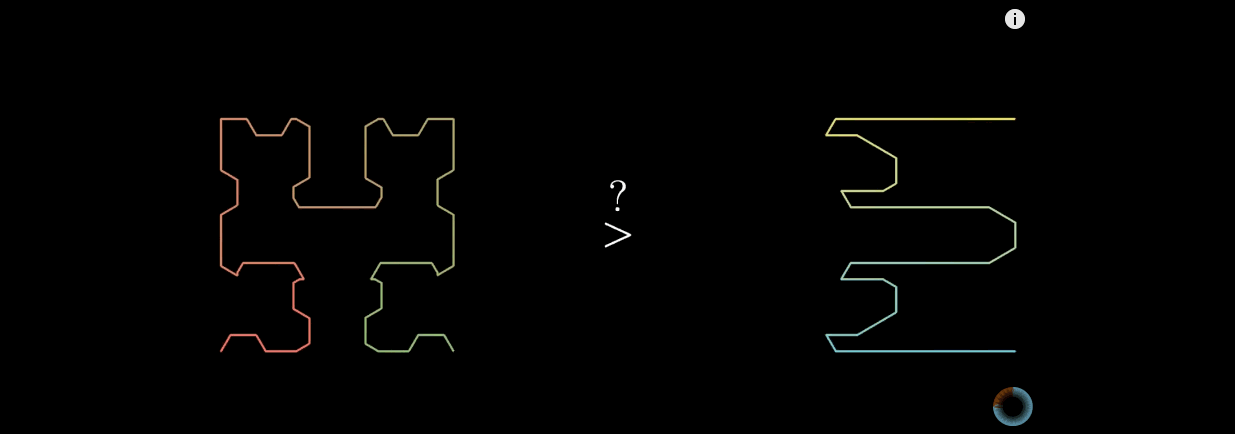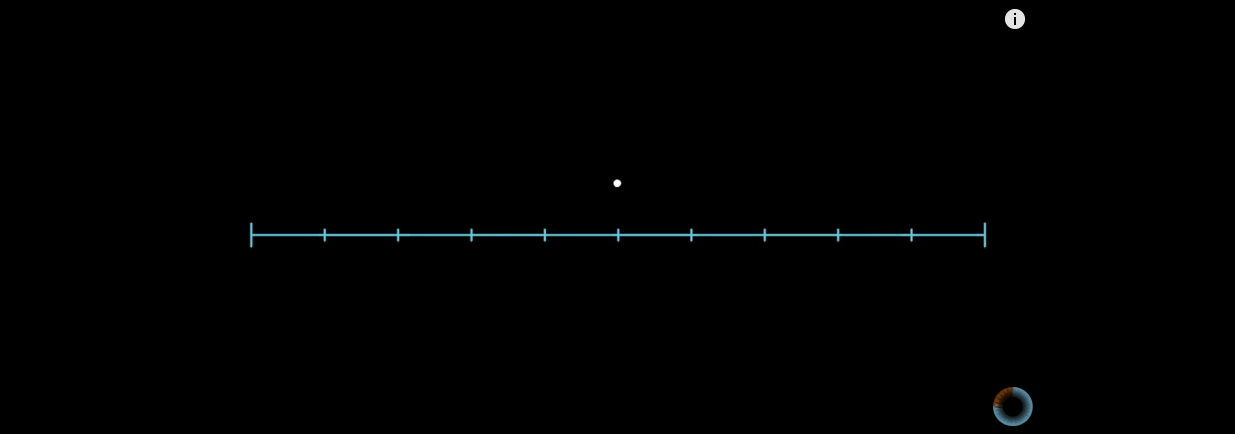#### (3) 连续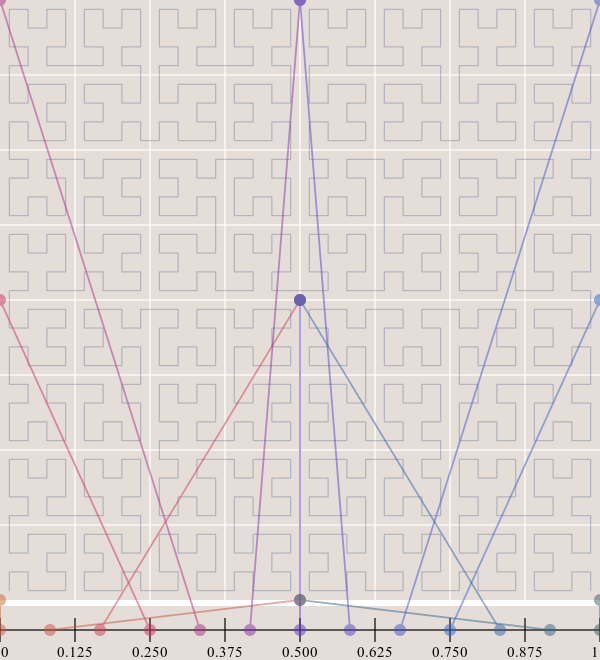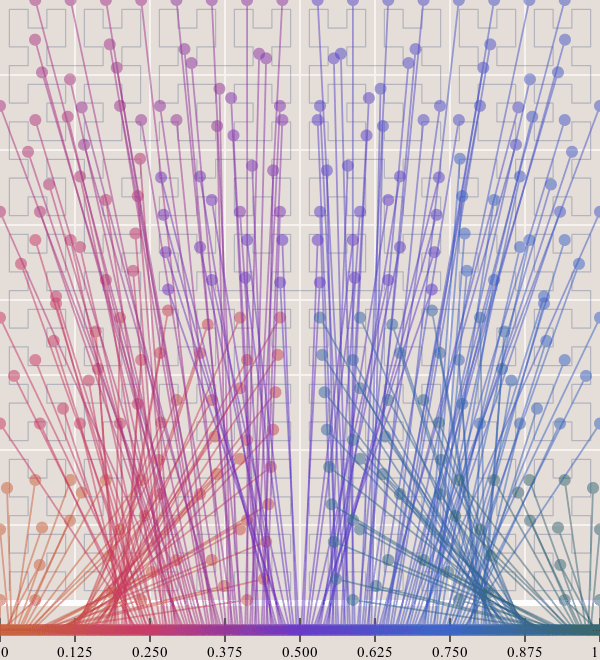## 四. S² 算法

Google’s S2 library is a real treasure, not only due to its capabilities for spatial indexing but also because it is a library that was released more than 4 years ago and it didn’t get the attention it deserved

### 1. 球面坐标转换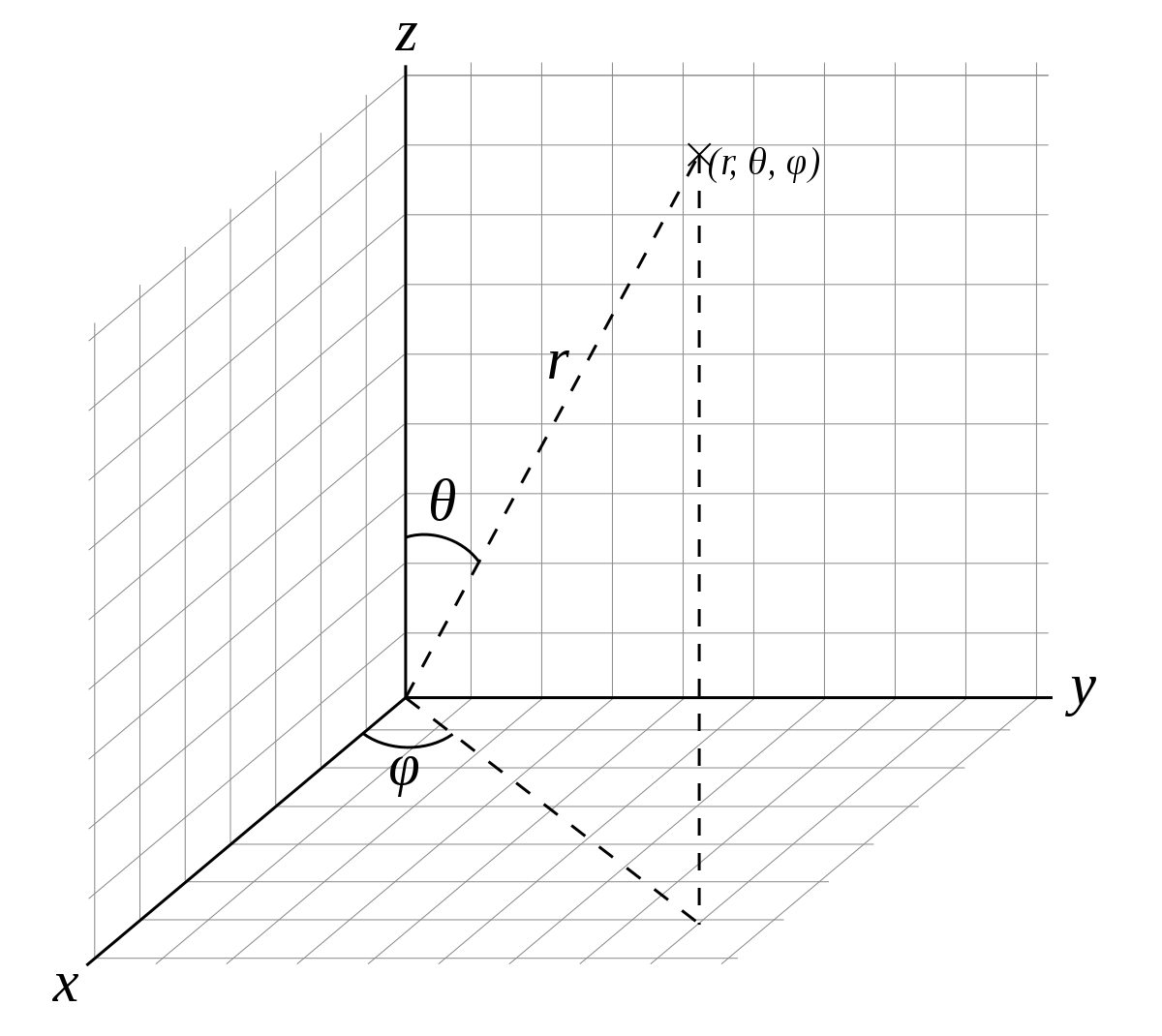vim

` x = r * sin θ * cos φ y = r * sin θ * sin φ  z = r * cos θ`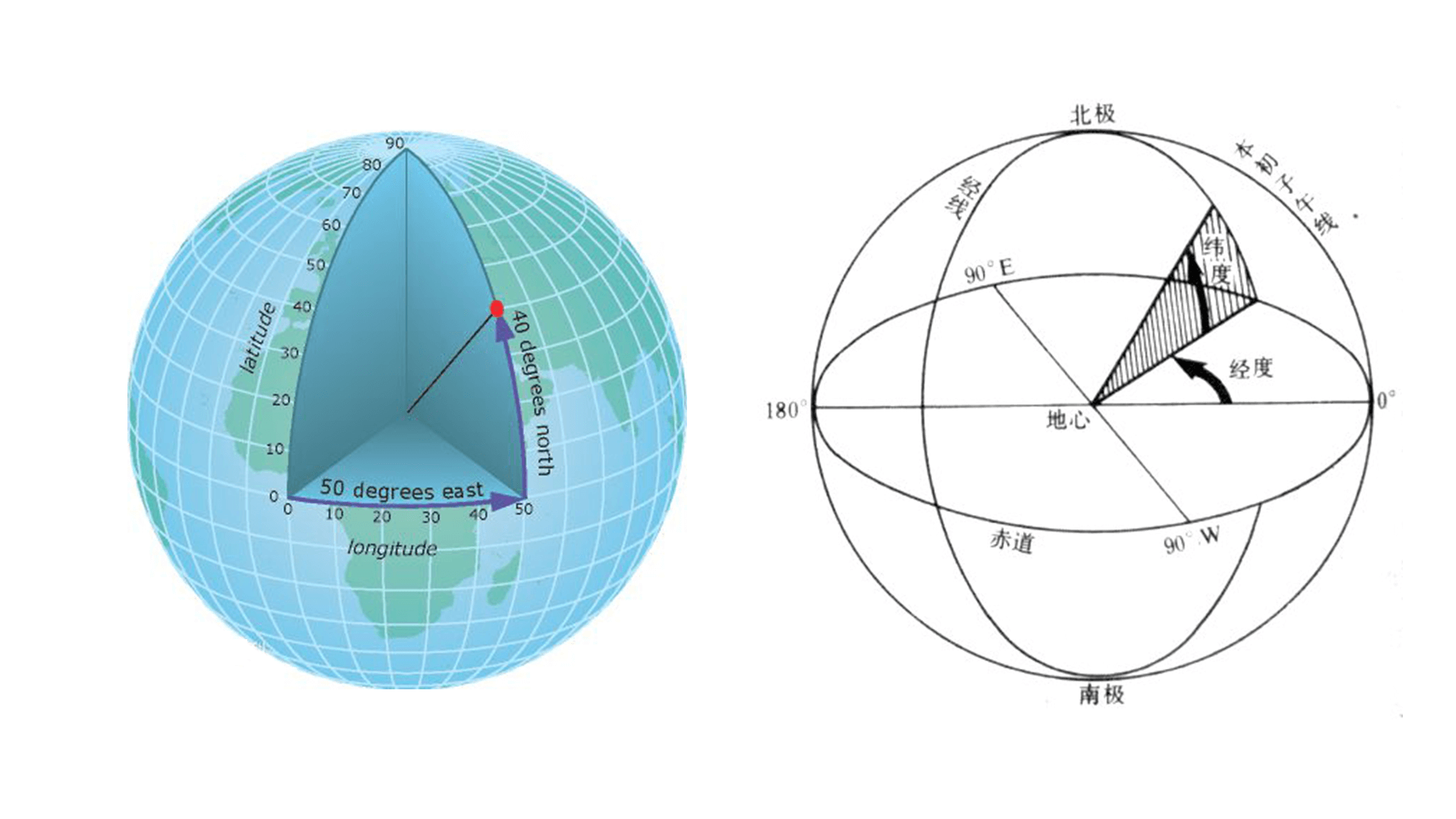### 2. 球面变平面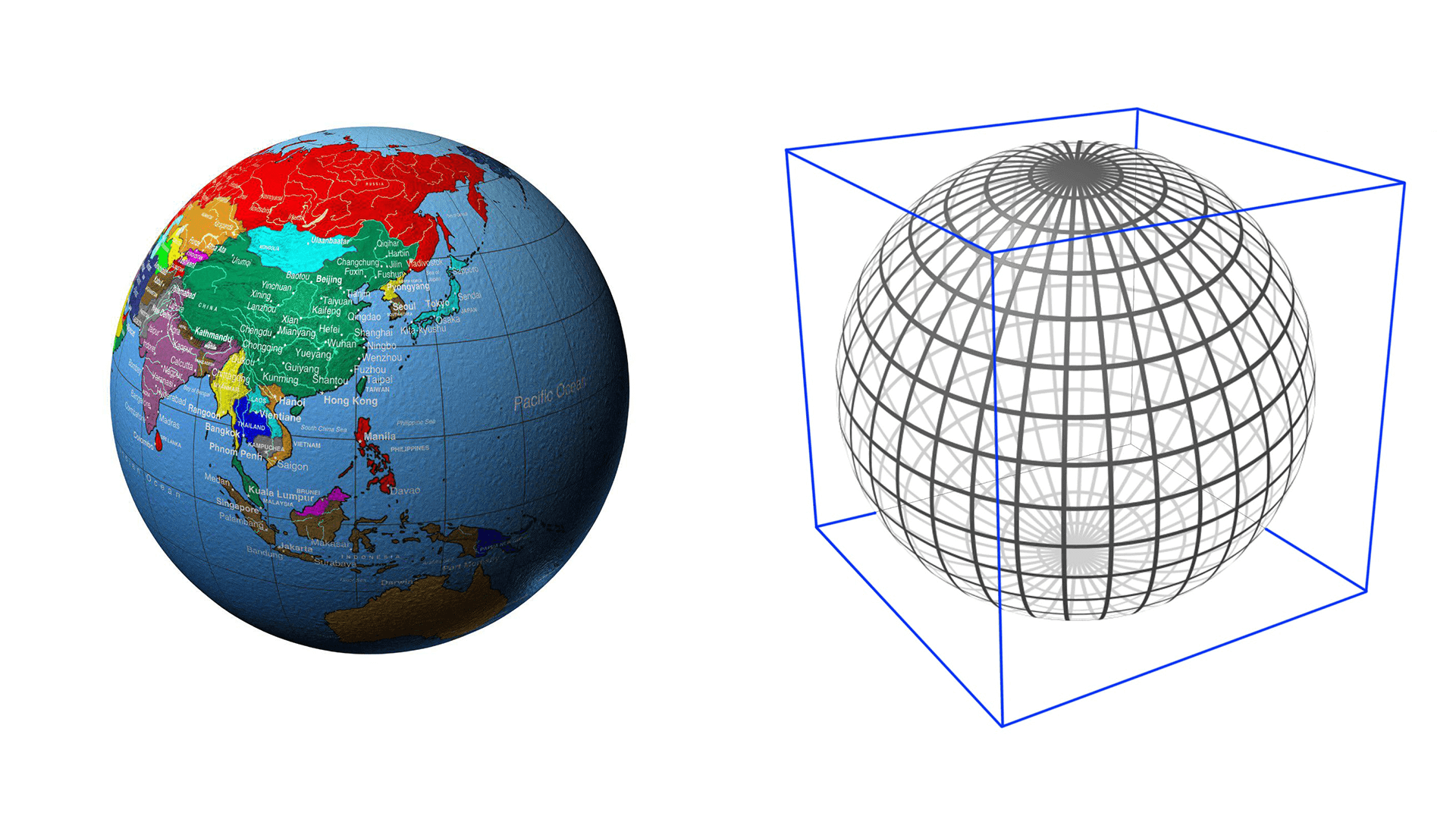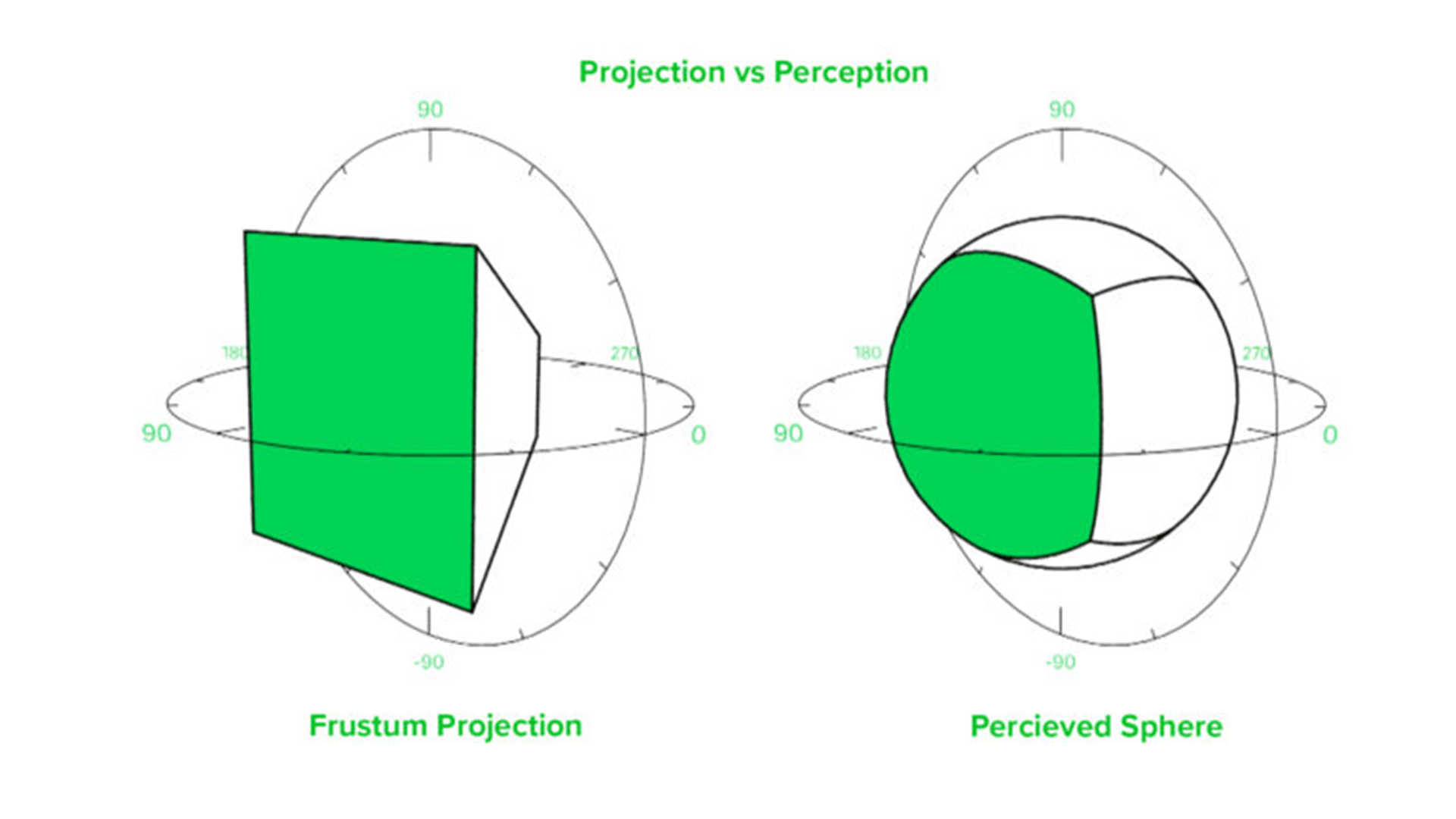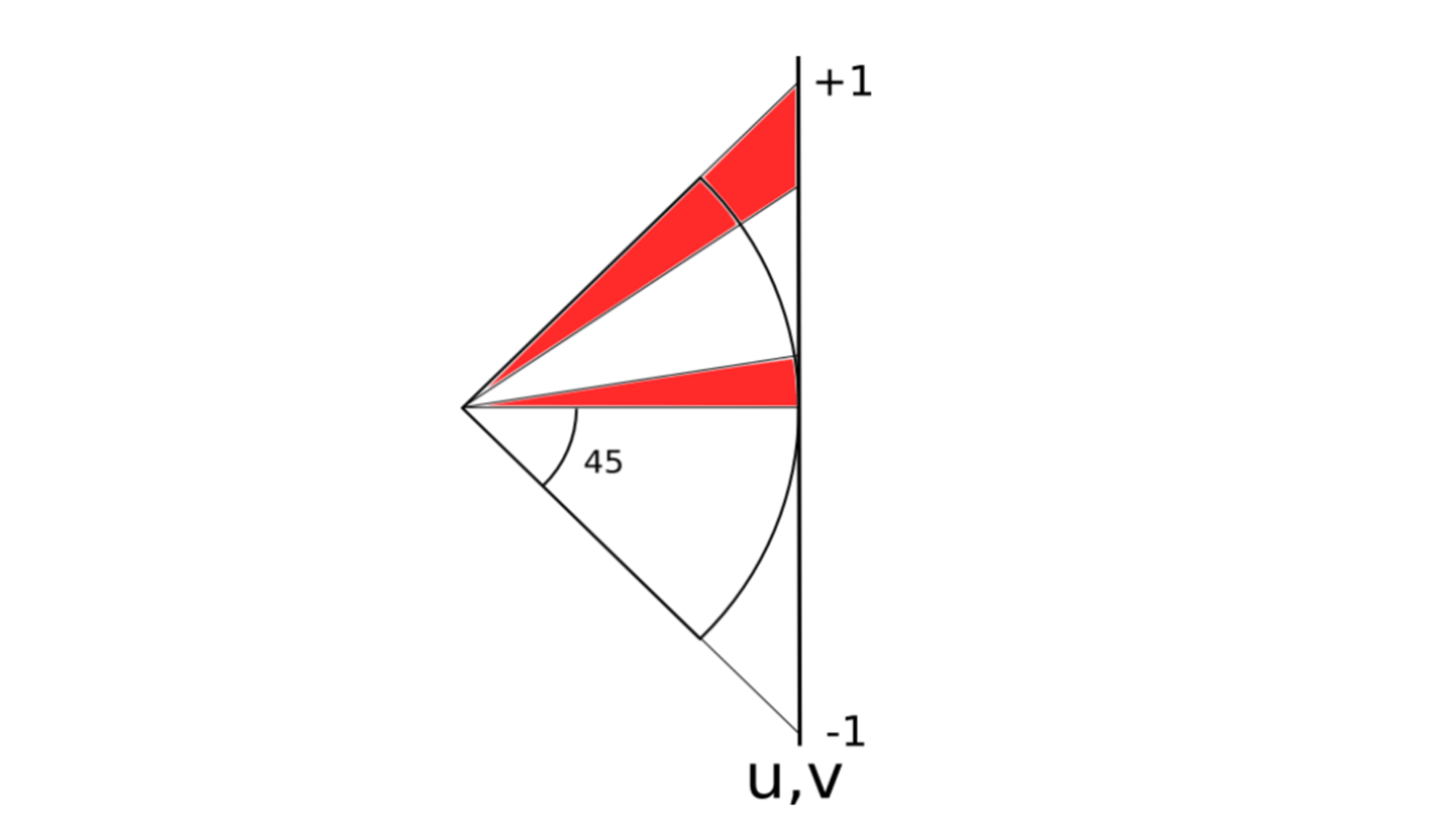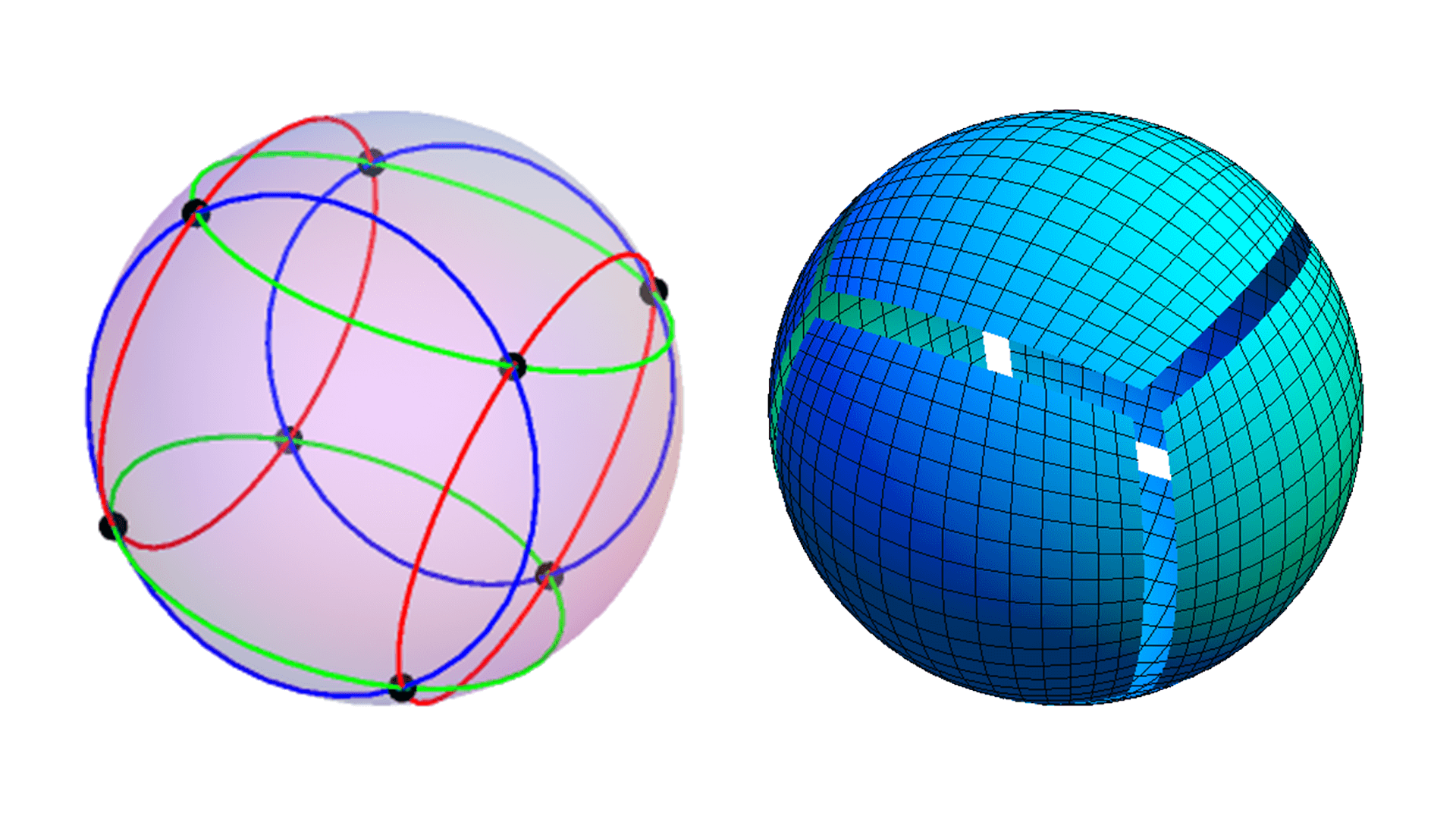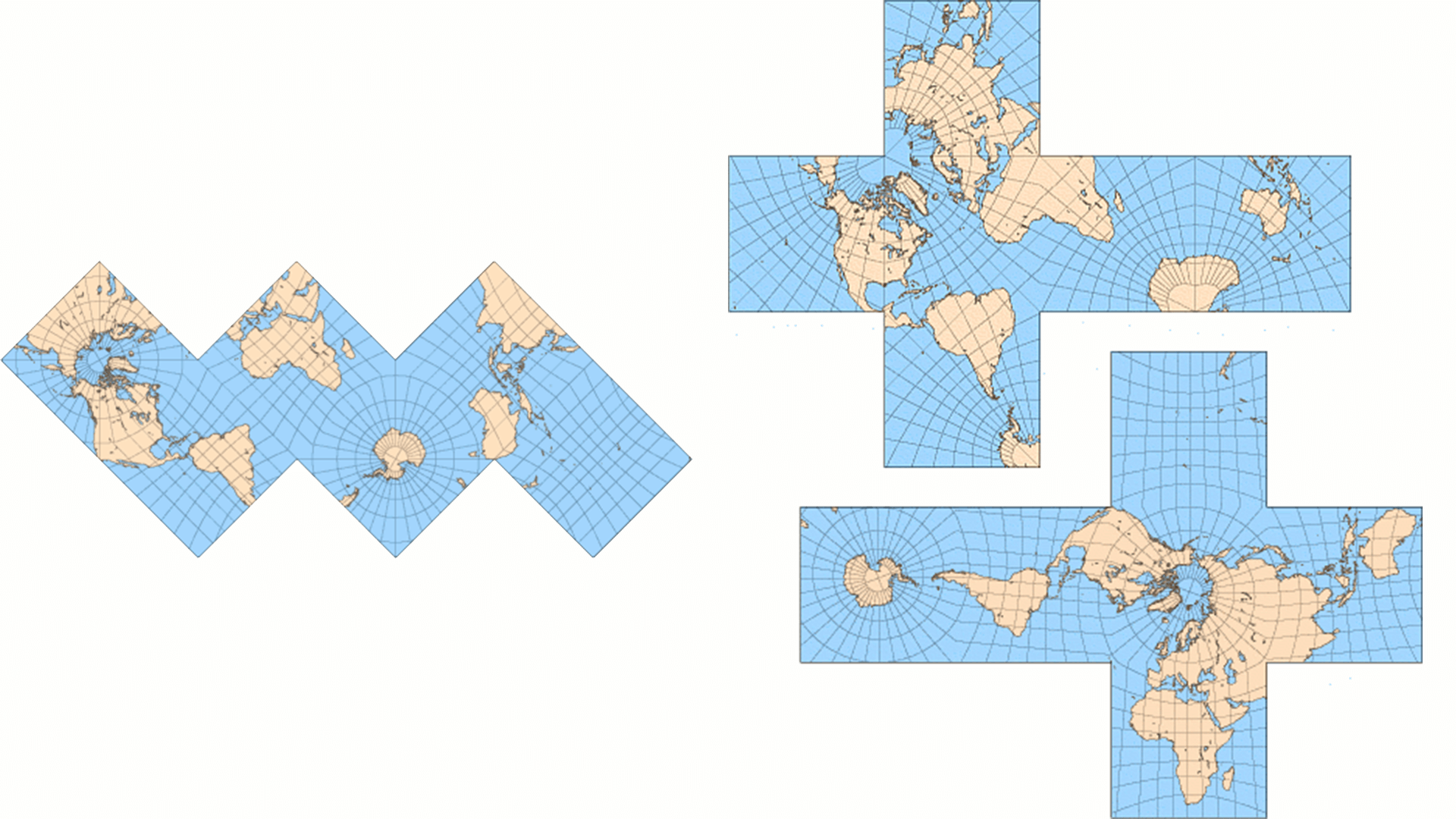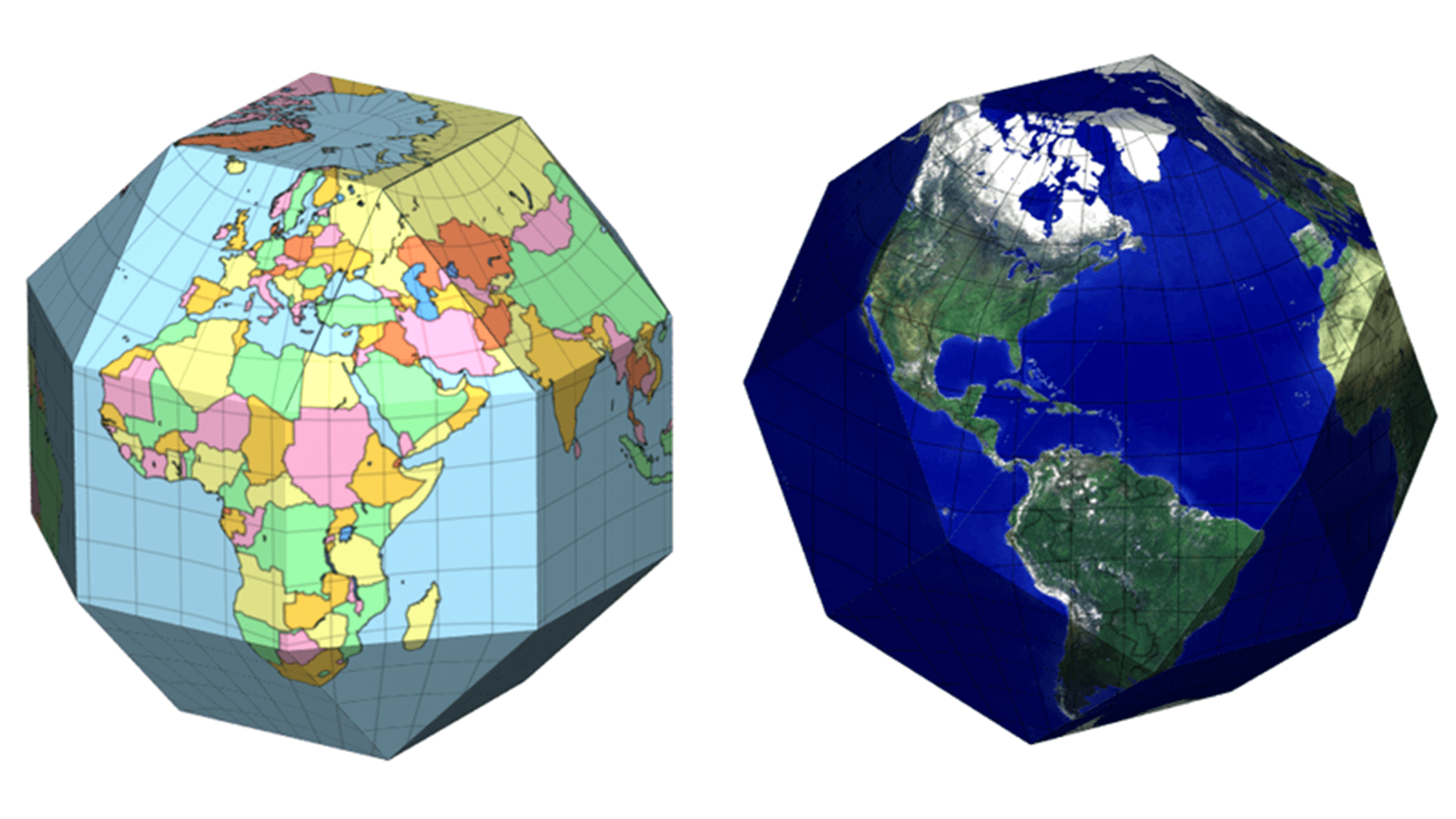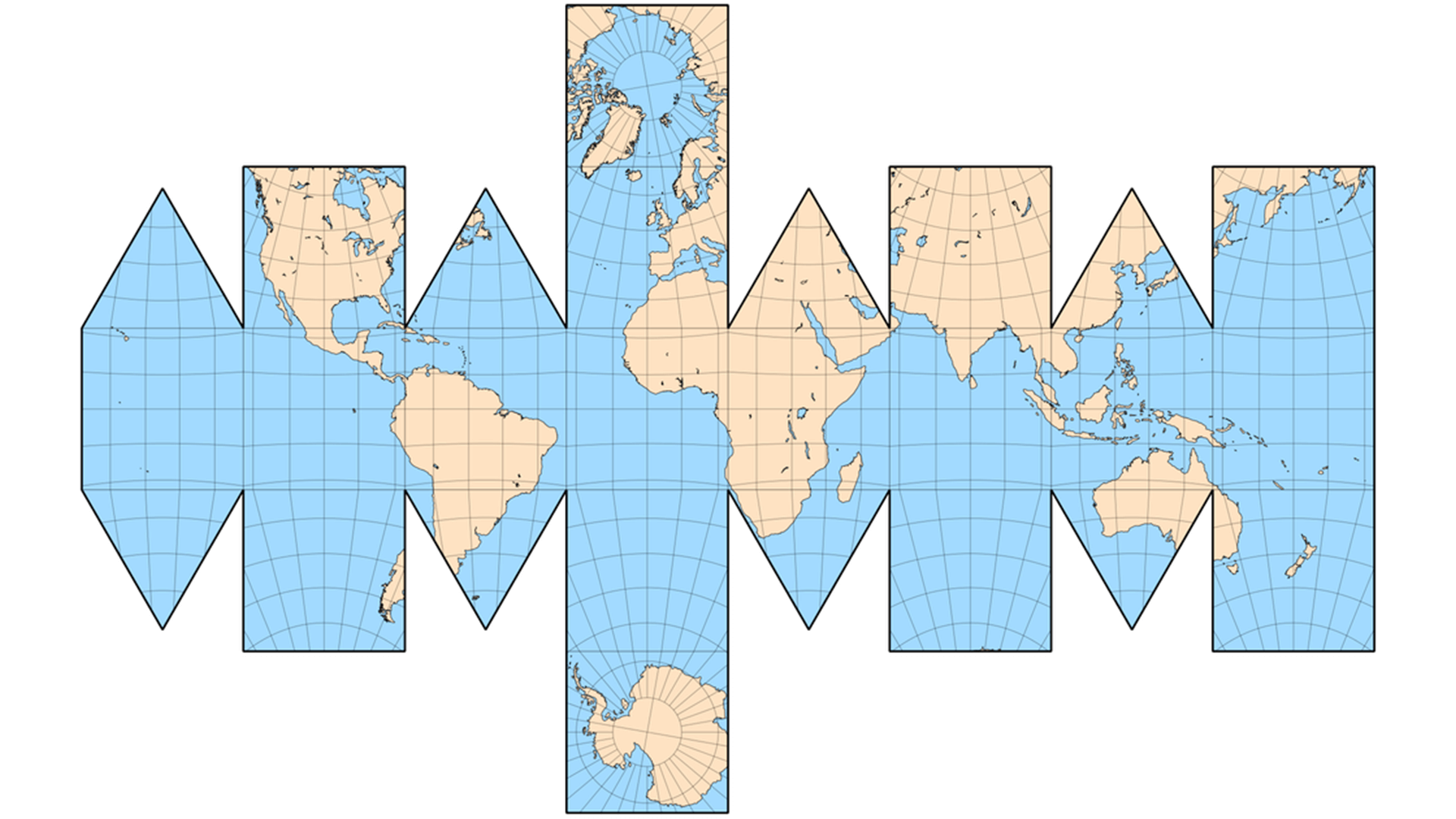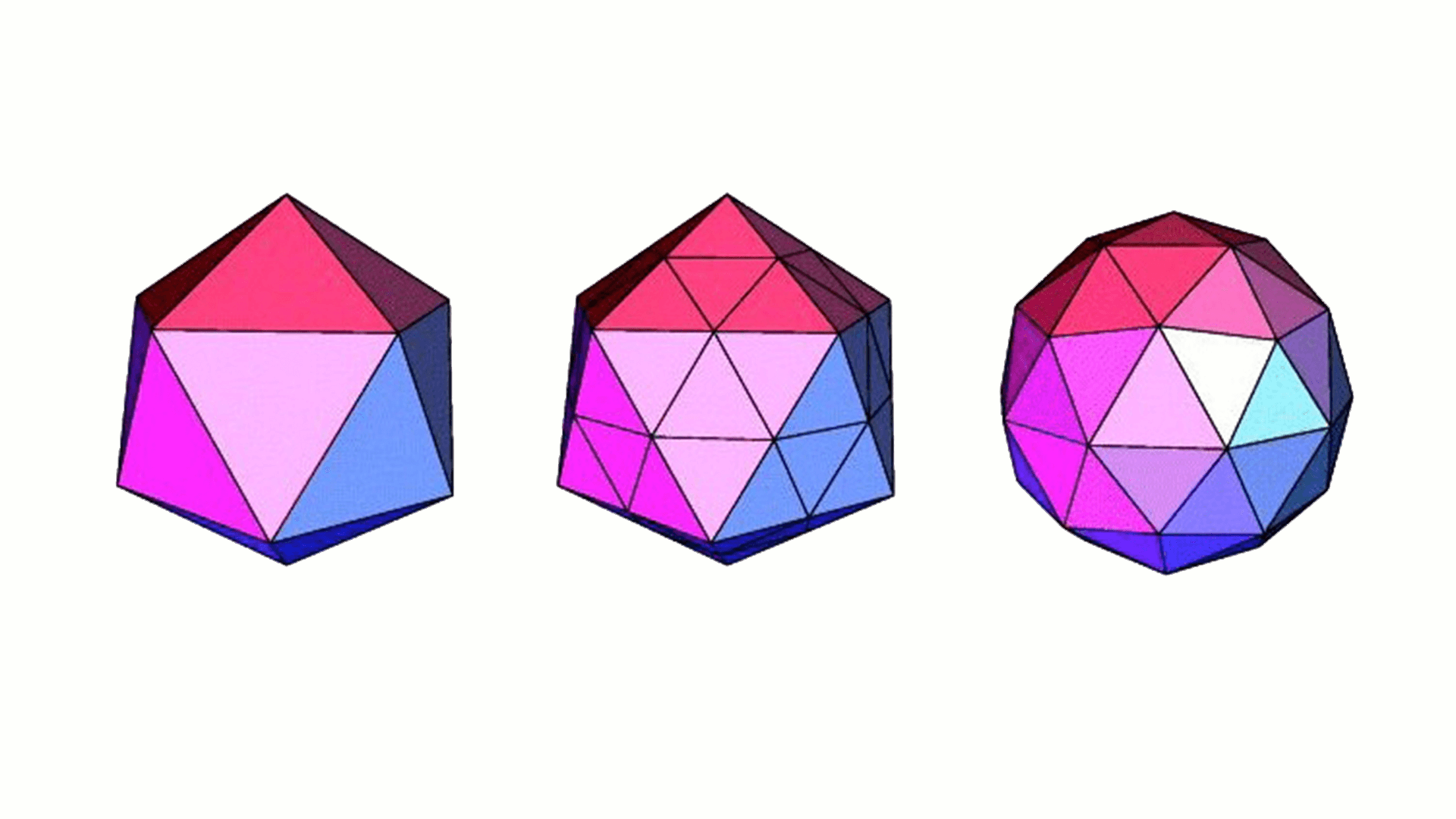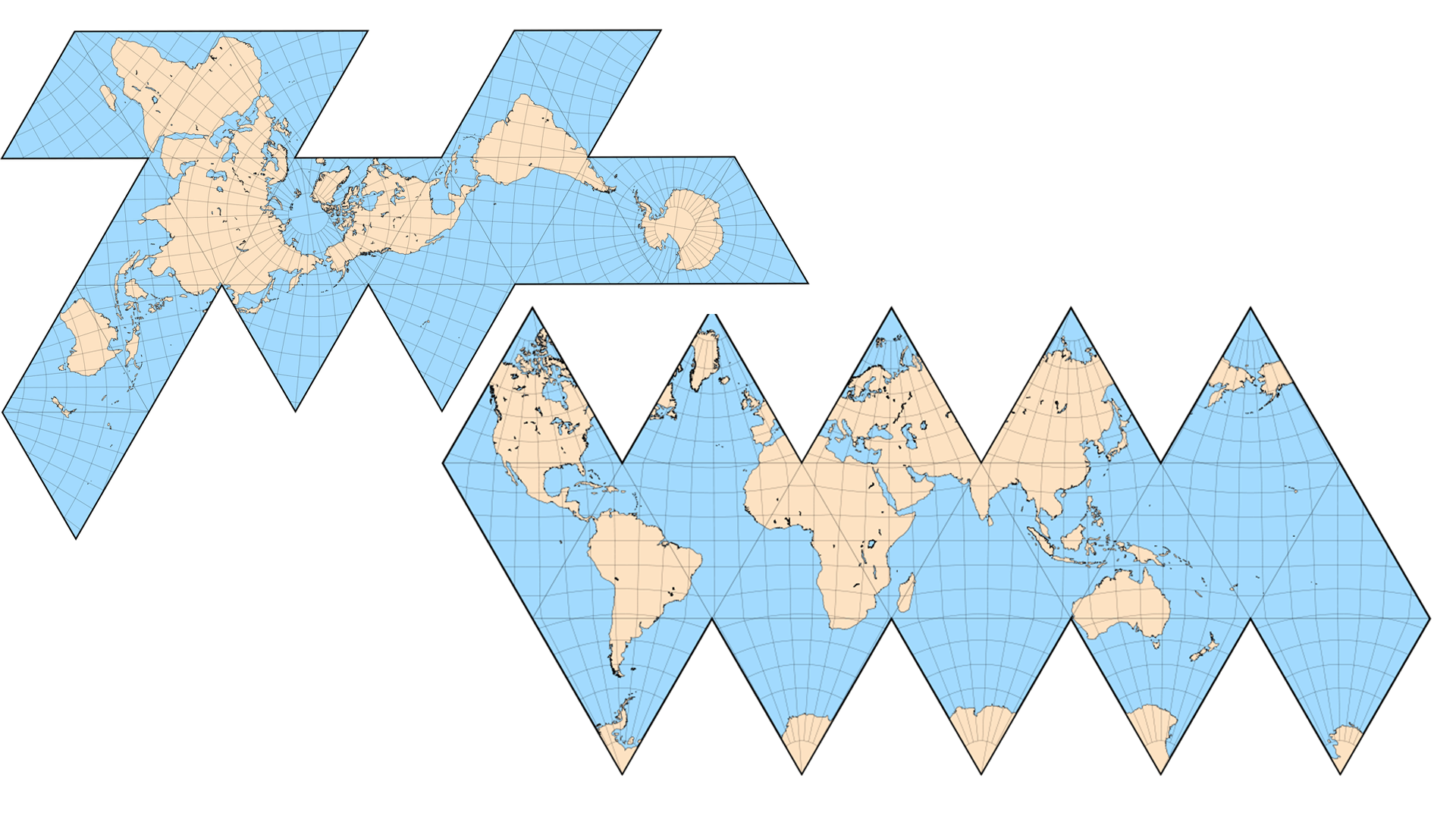20个三角形展开以后就可能变成这样。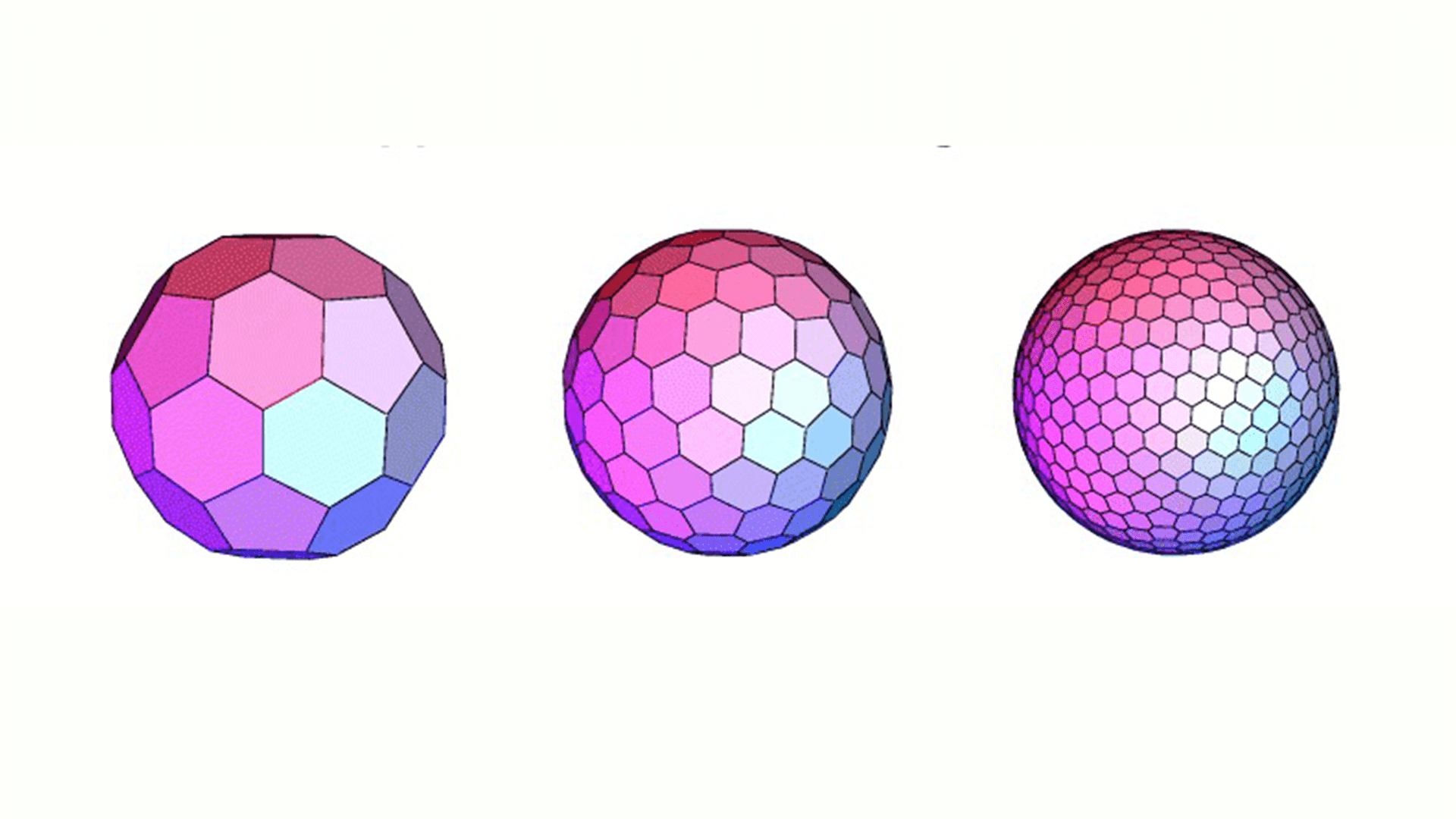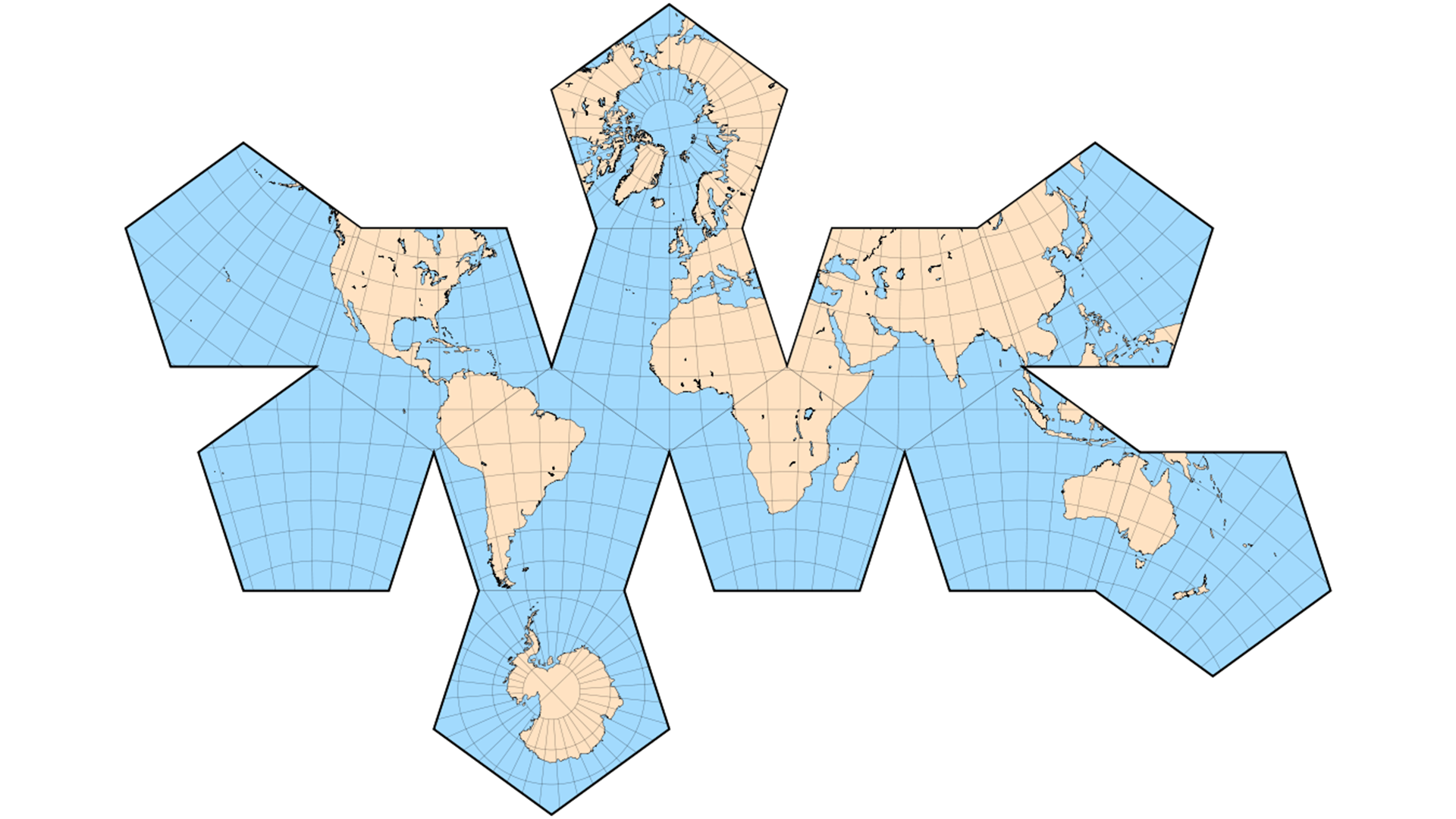Uber 在一个公开分享上提到了他们用的是六边形的网格，把城市划分为很多六边形。这块应该是他们自己开发的。也许滴滴也是划分六边形，也许滴滴有更好的划分方案也说不定。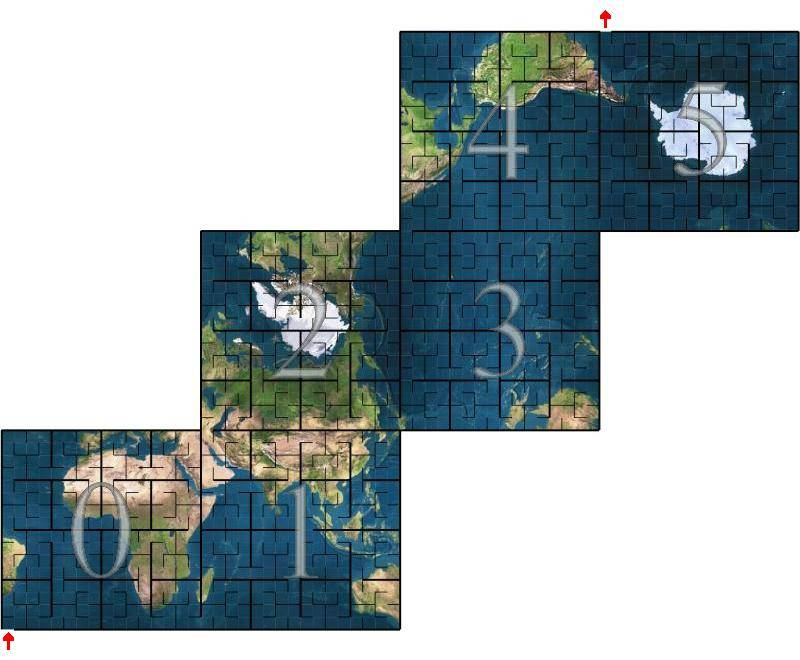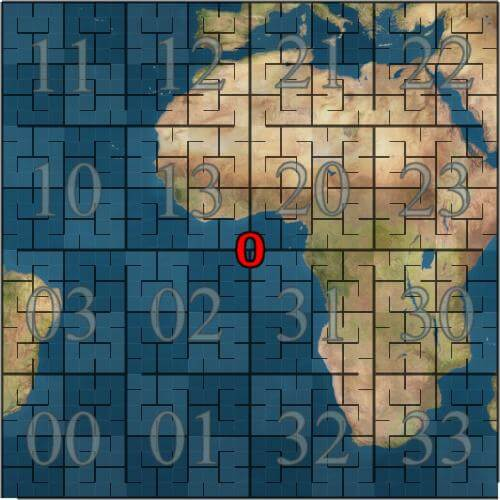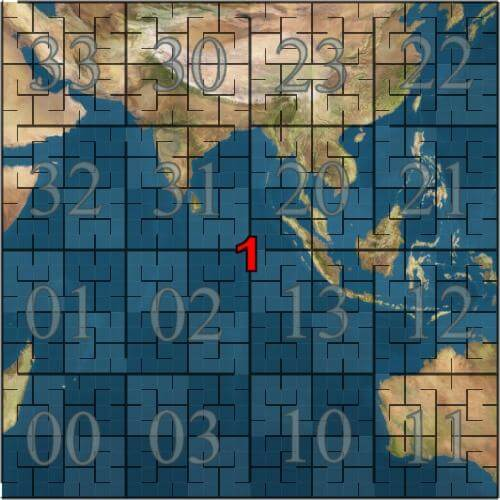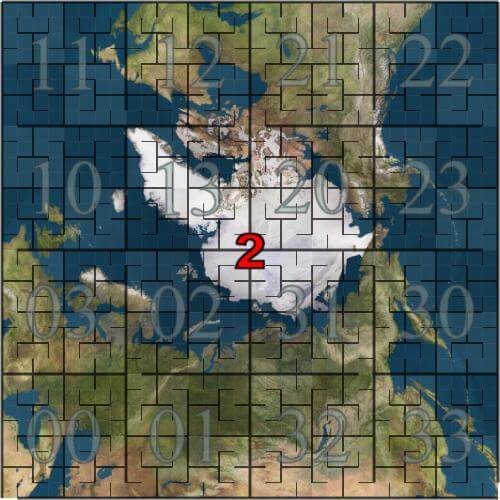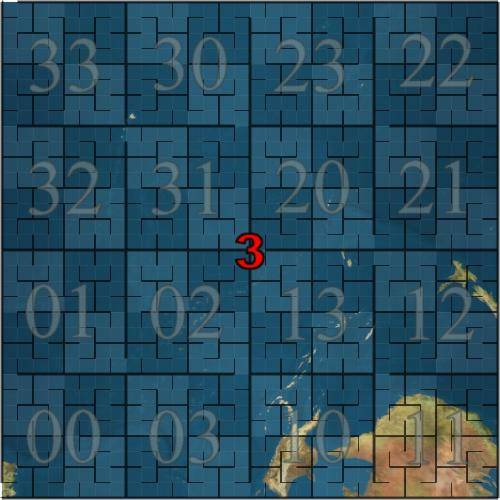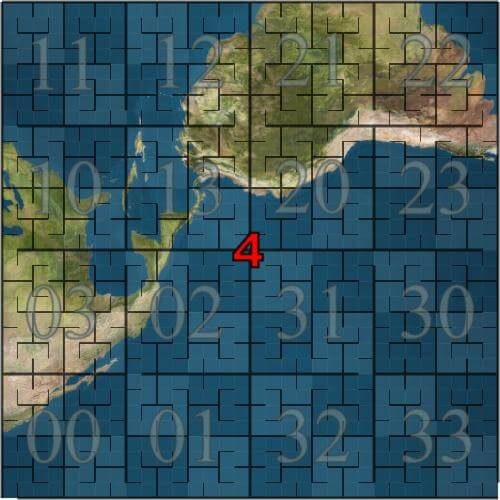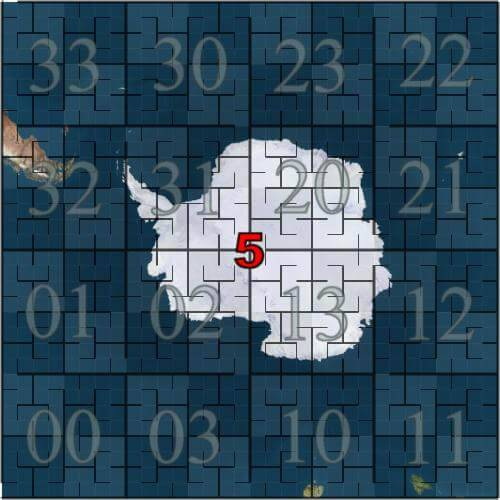### 3. 球面矩形投影修正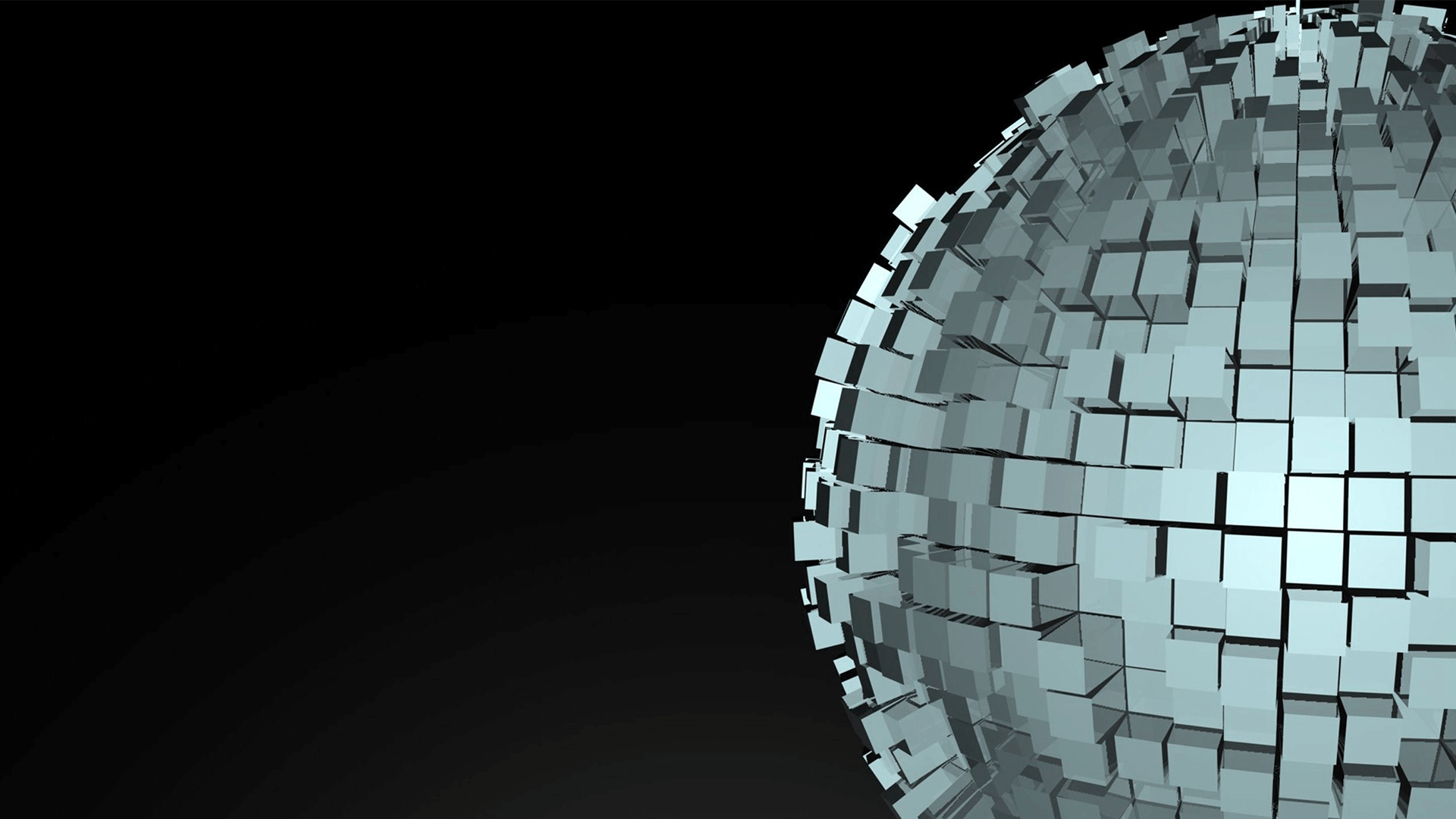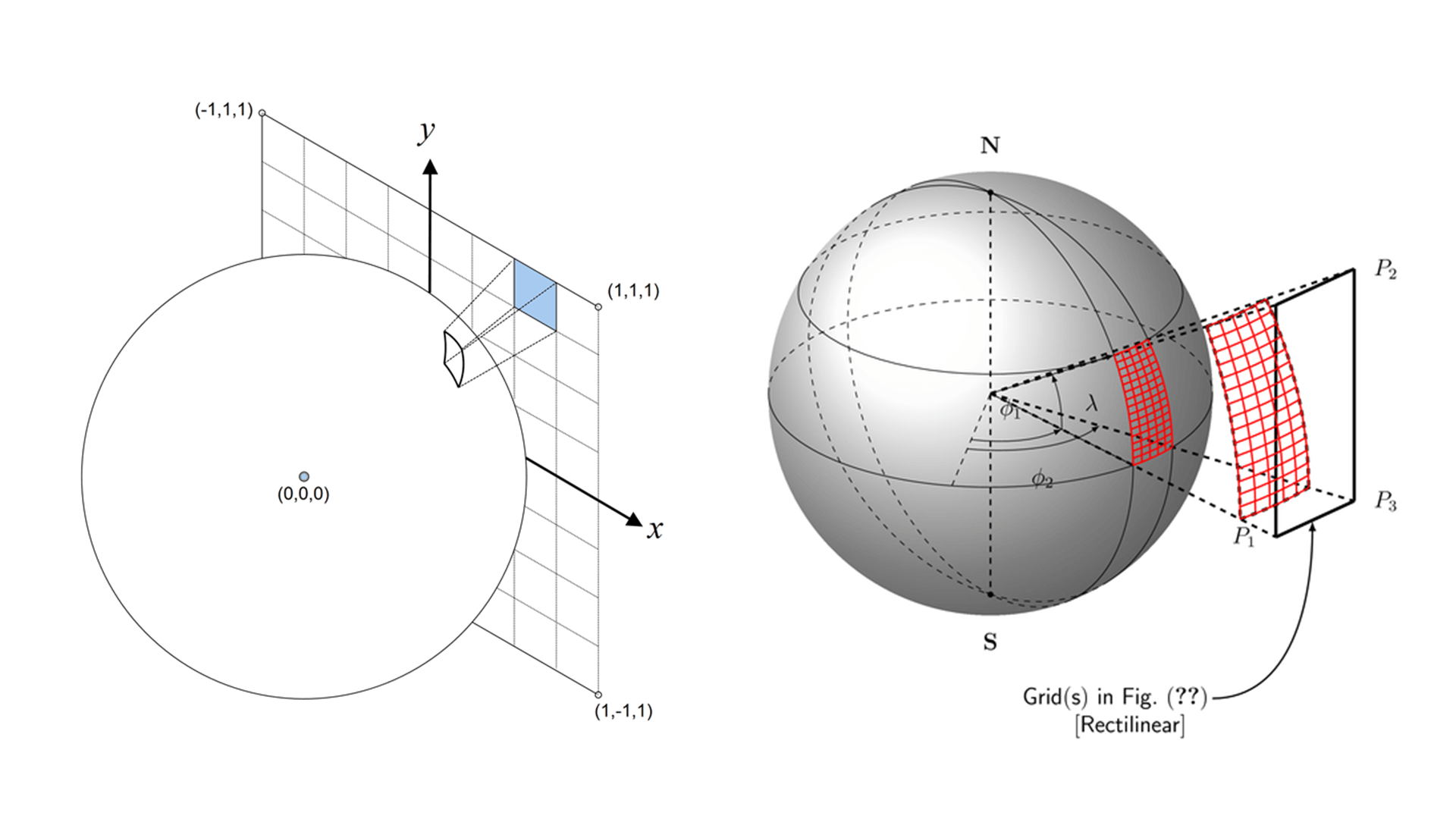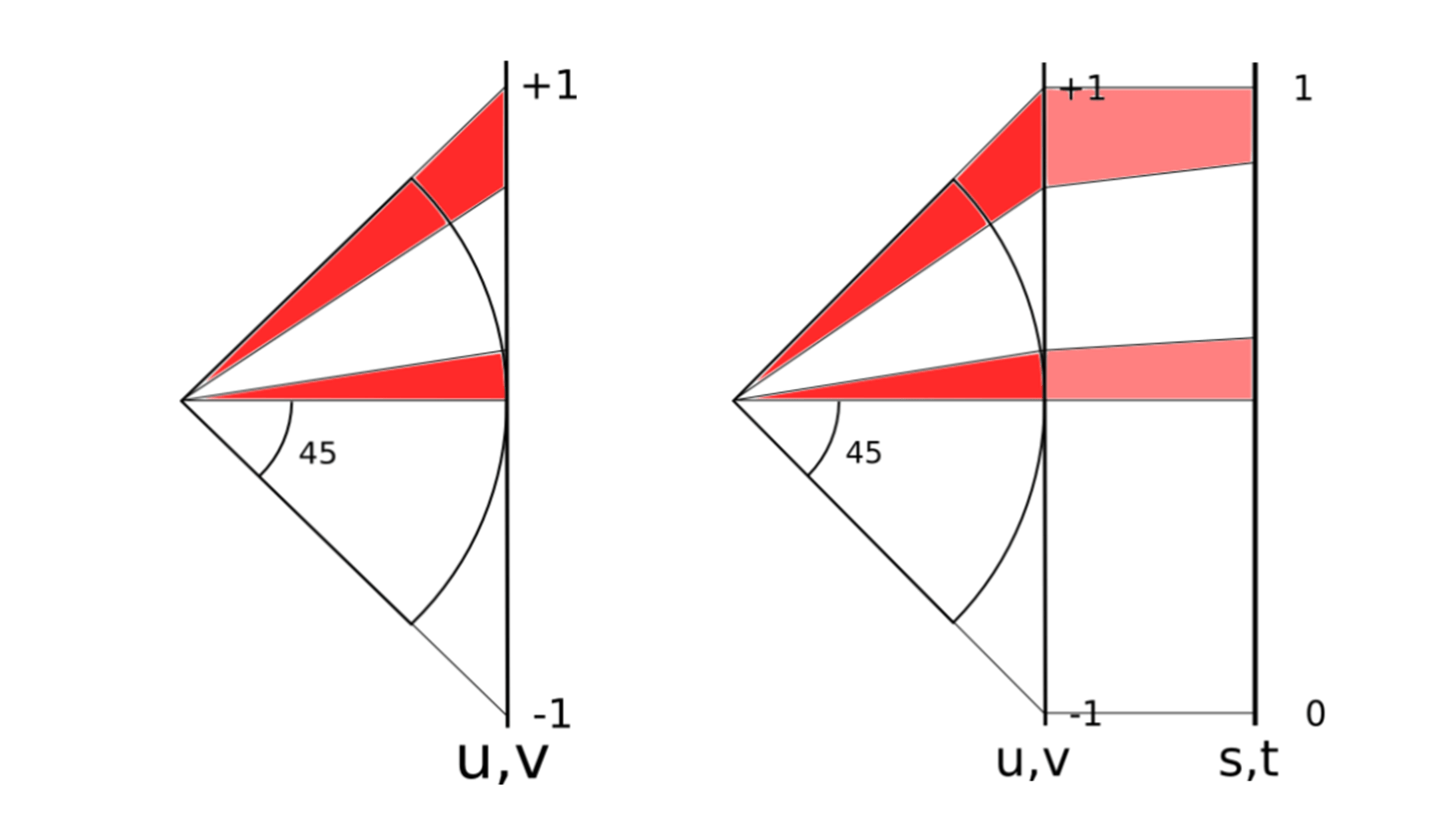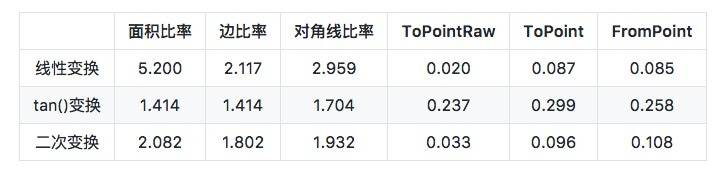C

` #define S2_PROJECTION S2_QUADRATIC_PROJECTION`

C

` #if S2_PROJECTION == S2_LINEAR_PROJECTION  inline double S2::STtoUV(double s) {   return 2 * s - 1; }  inline double S2::UVtoST(double u) {   return 0.5 * (u + 1); }  #elif S2_PROJECTION == S2_TAN_PROJECTION  inline double S2::STtoUV(double s) {   // Unfortunately, tan(M_PI_4) is slightly less than 1.0.  This isn't due to   // a flaw in the implementation of tan(), it's because the derivative of   // tan(x) at x=pi/4 is 2, and it happens that the two adjacent floating   // point numbers on either side of the infinite-precision value of pi/4 have   // tangents that are slightly below and slightly above 1.0 when rounded to   // the nearest double-precision result.    s = tan(M_PI_2 * s - M_PI_4);   return s + (1.0 / (GG_LONGLONG(1) << 53)) * s; }  inline double S2::UVtoST(double u) {   volatile double a = atan(u);   return (2 * M_1_PI) * (a + M_PI_4); }  #elif S2_PROJECTION == S2_QUADRATIC_PROJECTION  inline double S2::STtoUV(double s) {   if (s >= 0.5) return (1/3.) * (4*s*s - 1);   else          return (1/3.) * (1 - 4*(1-s)*(1-s)); }  inline double S2::UVtoST(double u) {   if (u >= 0) return 0.5 * sqrt(1 + 3*u);   else        return 1 - 0.5 * sqrt(1 - 3*u); }  #else  #error Unknown value for S2_PROJECTION  #endif`

C

` // 线性转换 u = 0.5 * ( u + 1)  // tan() 变换 u = 2 / pi * (atan(u) + pi / 4) = 2 * atan(u) / pi + 0.5  // 二次变换 u >= 0，u = 0.5 * sqrt(1 + 3*u) u < 0， u = 1 - 0.5 * sqrt(1 - 3*u)`

Go

` // stToUV converts an s or t value to the corresponding u or v value. // This is a non-linear transformation from [-1,1] to [-1,1] that // attempts to make the cell sizes more uniform. // This uses what the C++ version calls 'the quadratic transform'. func stToUV(s float64) float64 {     if s >= 0.5 {         return (1 / 3.) * (4*s*s - 1)     }     return (1 / 3.) * (1 - 4*(1-s)*(1-s)) }  // uvToST is the inverse of the stToUV transformation. Note that it // is not always true that uvToST(stToUV(x)) == x due to numerical // errors. func uvToST(u float64) float64 {     if u >= 0 {         return 0.5 * math.Sqrt(1+3*u)     }     return 1 - 0.5*math.Sqrt(1-3*u) }`

### 4. 点与坐标轴点相互转换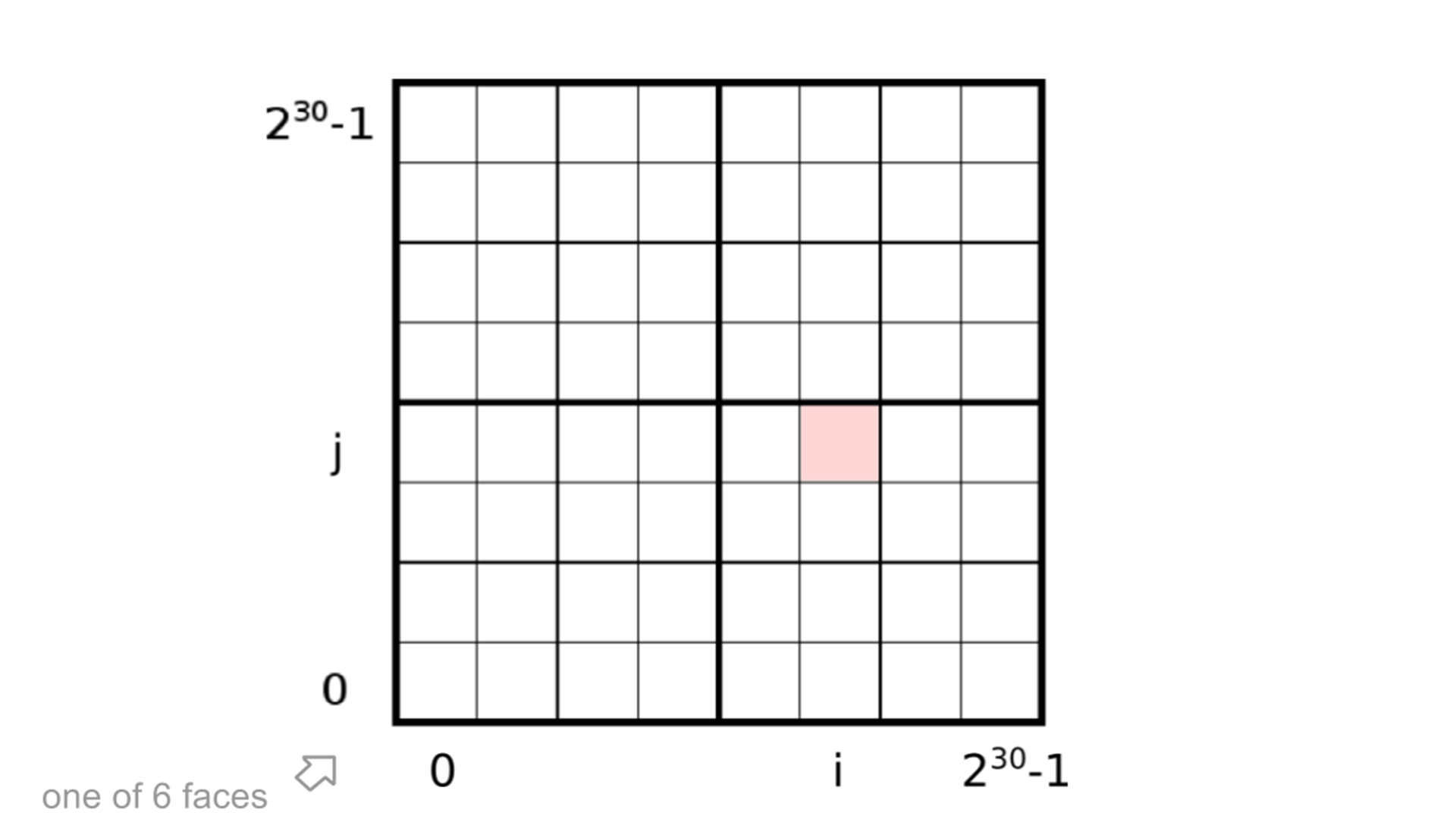s，t的值域是[0,1]，现在值域要扩大到[0,230-1]。

Go

` // stToIJ converts value in ST coordinates to a value in IJ coordinates. func stToIJ(s float64) int {     return clamp(int(math.Floor(maxSize*s)), 0, maxSize-1) }`

C ++ 的实现版本也一样

C

` inline int S2CellId::STtoIJ(double s) {   // Converting from floating-point to integers via static_cast is very slow   // on Intel processors because it requires changing the rounding mode.   // Rounding to the nearest integer using FastIntRound() is much faster.   // 这里减去0.5是为了四舍五入   return max(0, min(kMaxSize - 1, MathUtil::FastIntRound(kMaxSize * s - 0.5))); }`

### 5. 坐标轴点与希尔伯特曲线 Cell ID 相互转换

Go

` const (     lookupBits = 4     swapMask   = 0x01     invertMask = 0x02 )  var (     ijToPos = int{         {0, 1, 3, 2}, // canonical order         {0, 3, 1, 2}, // axes swapped         {2, 3, 1, 0}, // bits inverted         {2, 1, 3, 0}, // swapped & inverted     }     posToIJ = int{         {0, 1, 3, 2}, // canonical order:    (0,0), (0,1), (1,1), (1,0)         {0, 2, 3, 1}, // axes swapped:       (0,0), (1,0), (1,1), (0,1)         {3, 2, 0, 1}, // bits inverted:      (1,1), (1,0), (0,0), (0,1)         {3, 1, 0, 2}, // swapped & inverted: (1,1), (0,1), (0,0), (1,0)     }     posToOrientation = int{swapMask, 0, 0, invertMask | swapMask}     lookupIJ         [1 << (2*lookupBits + 2)]int     lookupPos        [1 << (2*lookupBits + 2)]int )`

posToIJ 代表的是一个矩阵，里面记录了一些单元希尔伯特曲线的位置信息。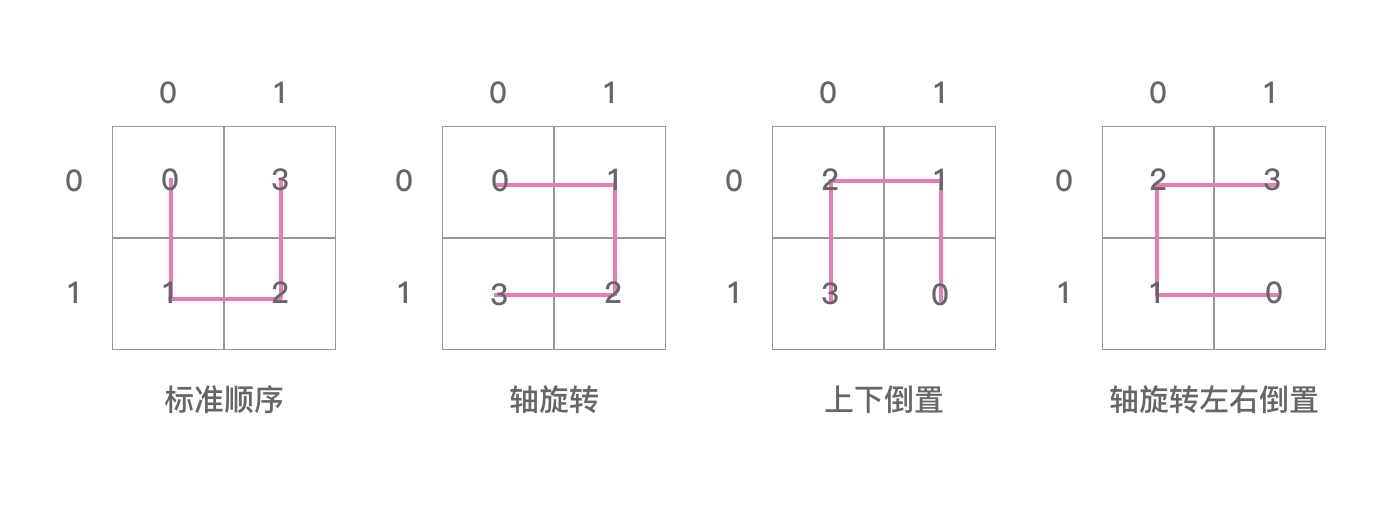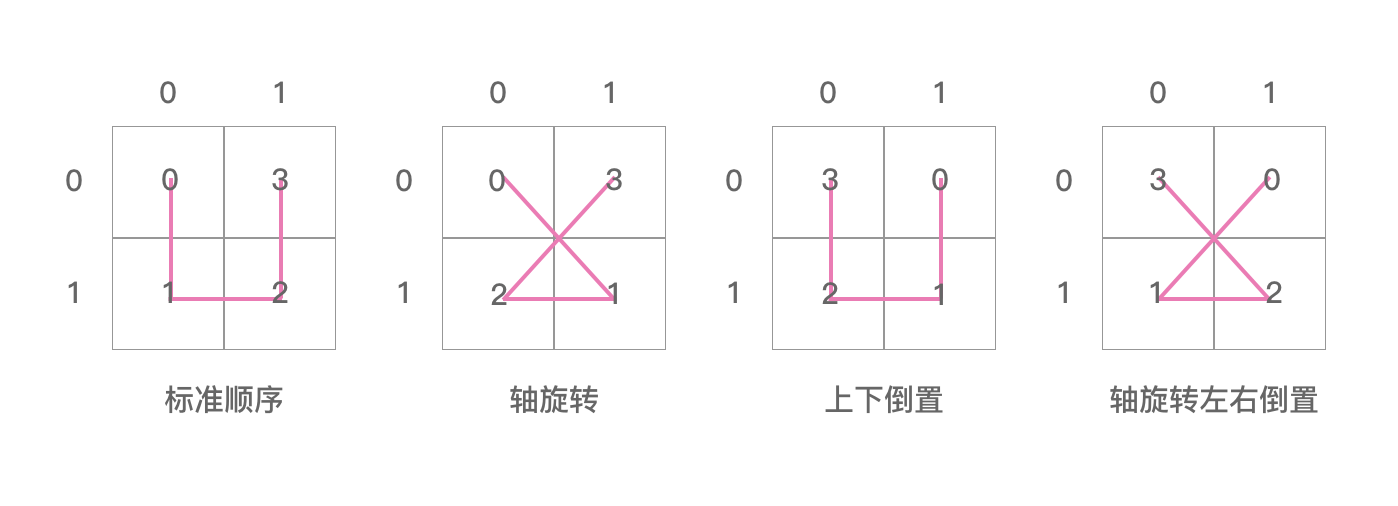posToOrientation 数组里面装了4个数字，分别是1,0,0,3。
lookupIJ 和 lookupPos 分别是两个容量为1024的数组。这里面分别对应的就是希尔伯特曲线 ID 转换成坐标轴 IJ 的转换表，和坐标轴 IJ 转换成希尔伯特曲线 ID 的转换表。

Go

` func init() {     initLookupCell(0, 0, 0, 0, 0, 0)     initLookupCell(0, 0, 0, swapMask, 0, swapMask)     initLookupCell(0, 0, 0, invertMask, 0, invertMask)     initLookupCell(0, 0, 0, swapMask|invertMask, 0, swapMask|invertMask) }`

Go

` // initLookupCell initializes the lookupIJ table at init time. func initLookupCell(level, i, j, origOrientation, pos, orientation int) {      if level == lookupBits {         ij := (i << lookupBits) + j         lookupPos[(ij<<2)+origOrientation] = (pos << 2) + orientation         lookupIJ[(pos<<2)+origOrientation] = (ij << 2) + orientation              return     }      level++     i <<= 1     j <<= 1     pos <<= 2          r := posToIJ[orientation]          initLookupCell(level, i+(r>>1), j+(r&1), origOrientation, pos, orientation^posToOrientation)     initLookupCell(level, i+(r>>1), j+(r&1), origOrientation, pos+1, orientation^posToOrientation)     initLookupCell(level, i+(r>>1), j+(r&1), origOrientation, pos+2, orientation^posToOrientation)     initLookupCell(level, i+(r>>1), j+(r&1), origOrientation, pos+3, orientation^posToOrientation) }`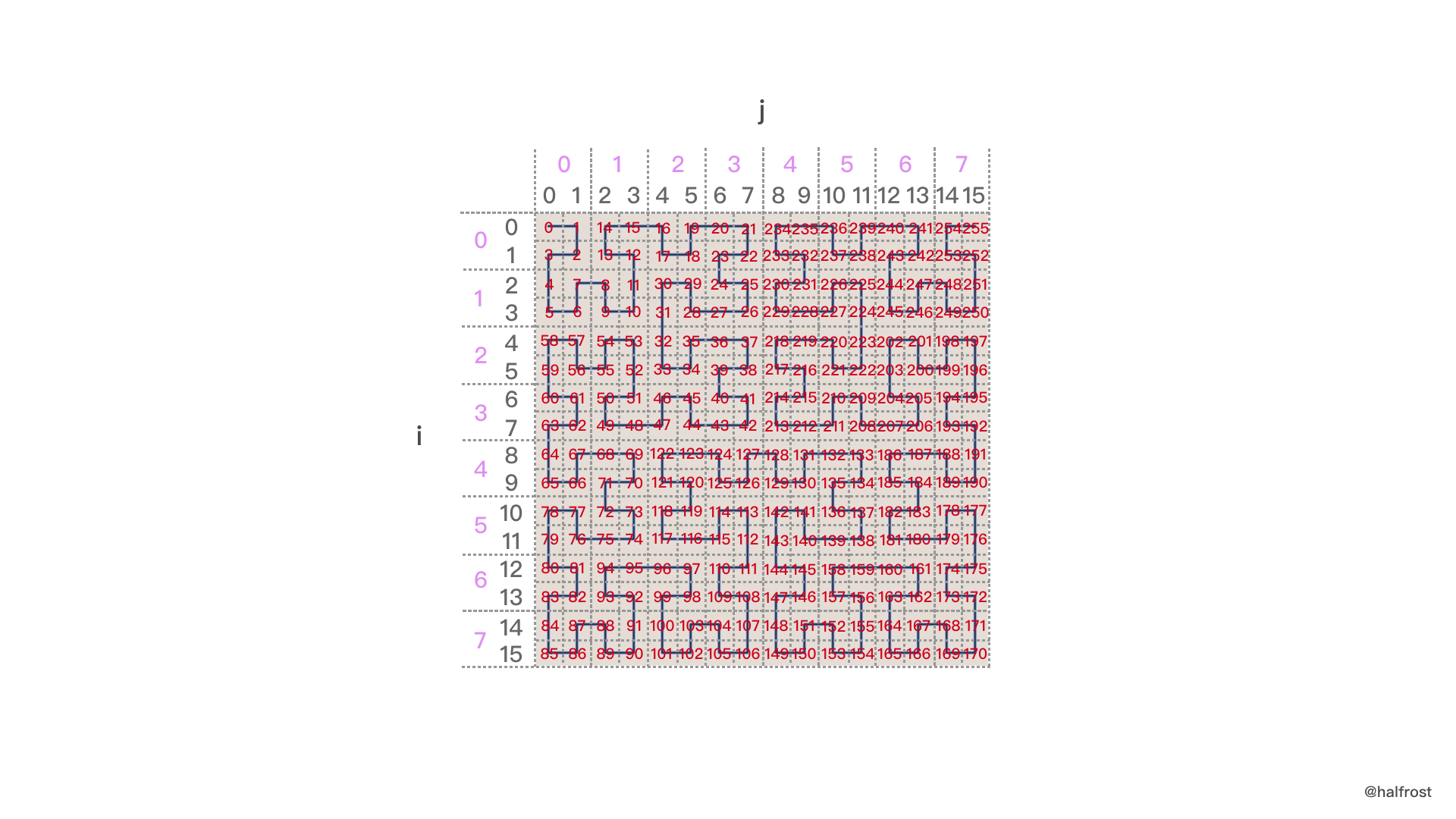lookupPos 表计算过程。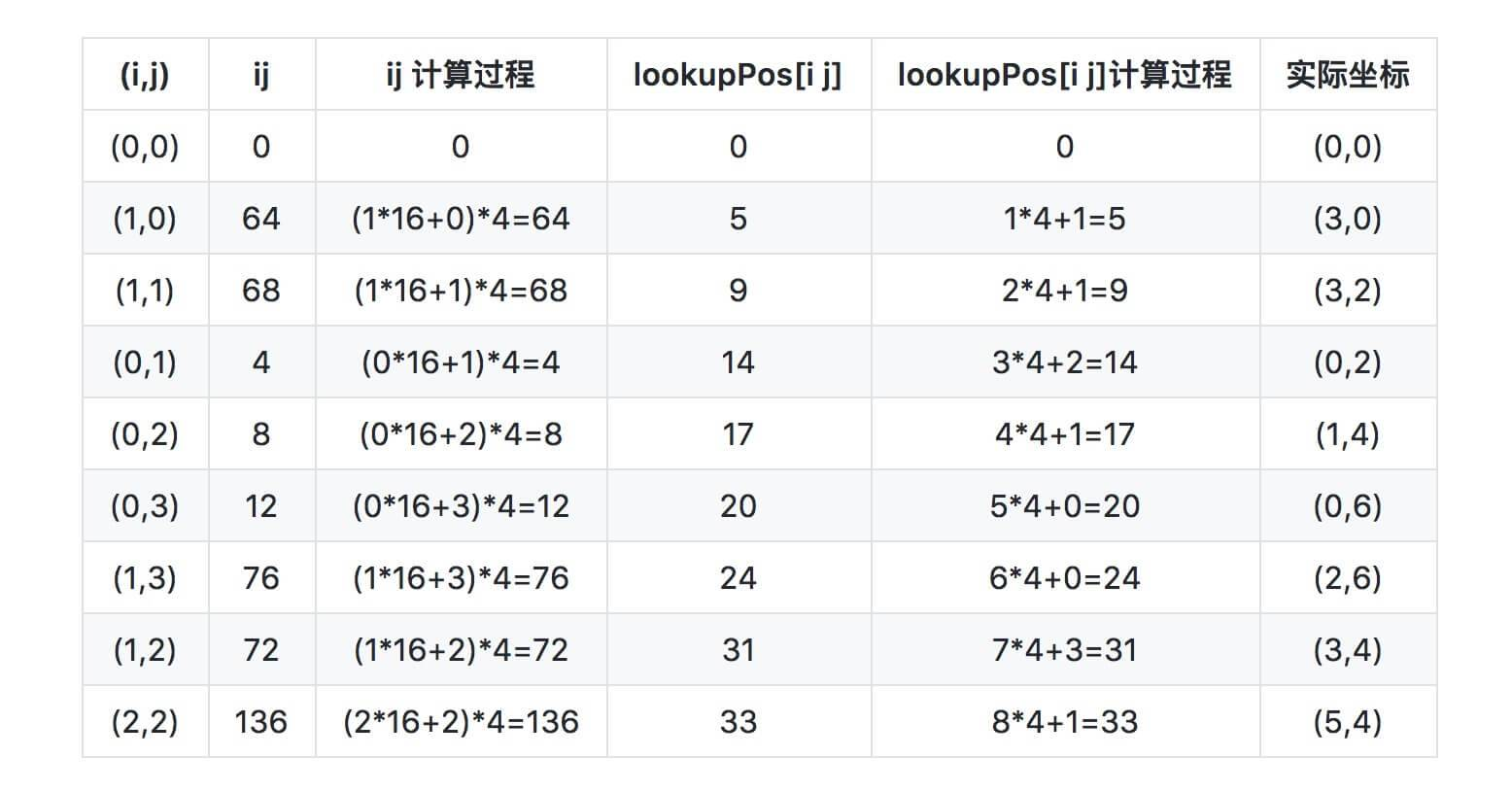lookupIJ 数组里面记录了反向的信息。lookupIJ 数组 和 lookupPos 数组存储的信息正好是反向的。lookupIJ 数组 下表存的值是 lookupPos 数组 的下表。我们查 lookupIJ 数组 ，lookupIJ的值就是8，对应算出来(i,j)=(0,2)。这个时候的i，j还是大格子。还是需要借助 posToIJ 数组 里面描述的形状信息。当前形状是轴旋转，之前也知道 orientation = 1，由于每个坐标里面有4个小格子，所以一个i，j代表的是2个小格子，所以需要乘以2，再加上形状信息里面的方向，可以计算出实际的坐标 (0 * 2 + 1 , 2 * 2 + 0) = ( 1，4) 。

### 6. S2 Cell ID 数据结构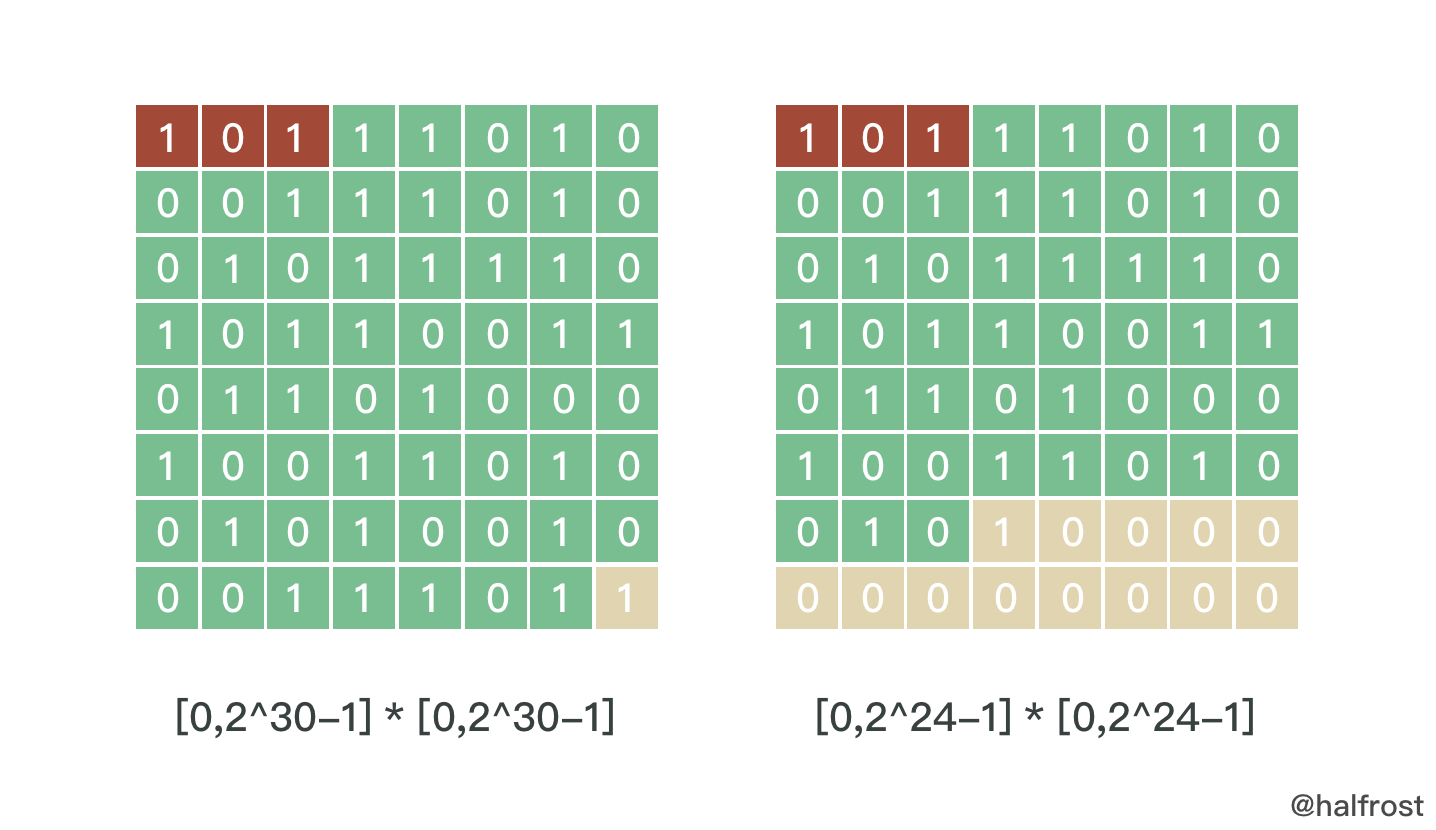64位的最后一位是1，这一位是特意留出来的。用来快速查找中间有多少位。从末尾最后一位向前查找，找到第一个不为0的位置，即找到第一个1。这一位的前一位到开头的第4位（因为前3位被占用）都是可用数字。

C

` MinAreaMetric = Metric{2, 8 * math.Sqrt2 / 9}  AvgAreaMetric = Metric{2, 4 * math.Pi / 6}  MaxAreaMetric = Metric{2, 2.635799256963161491}`

(下图单位是km2，平方公里)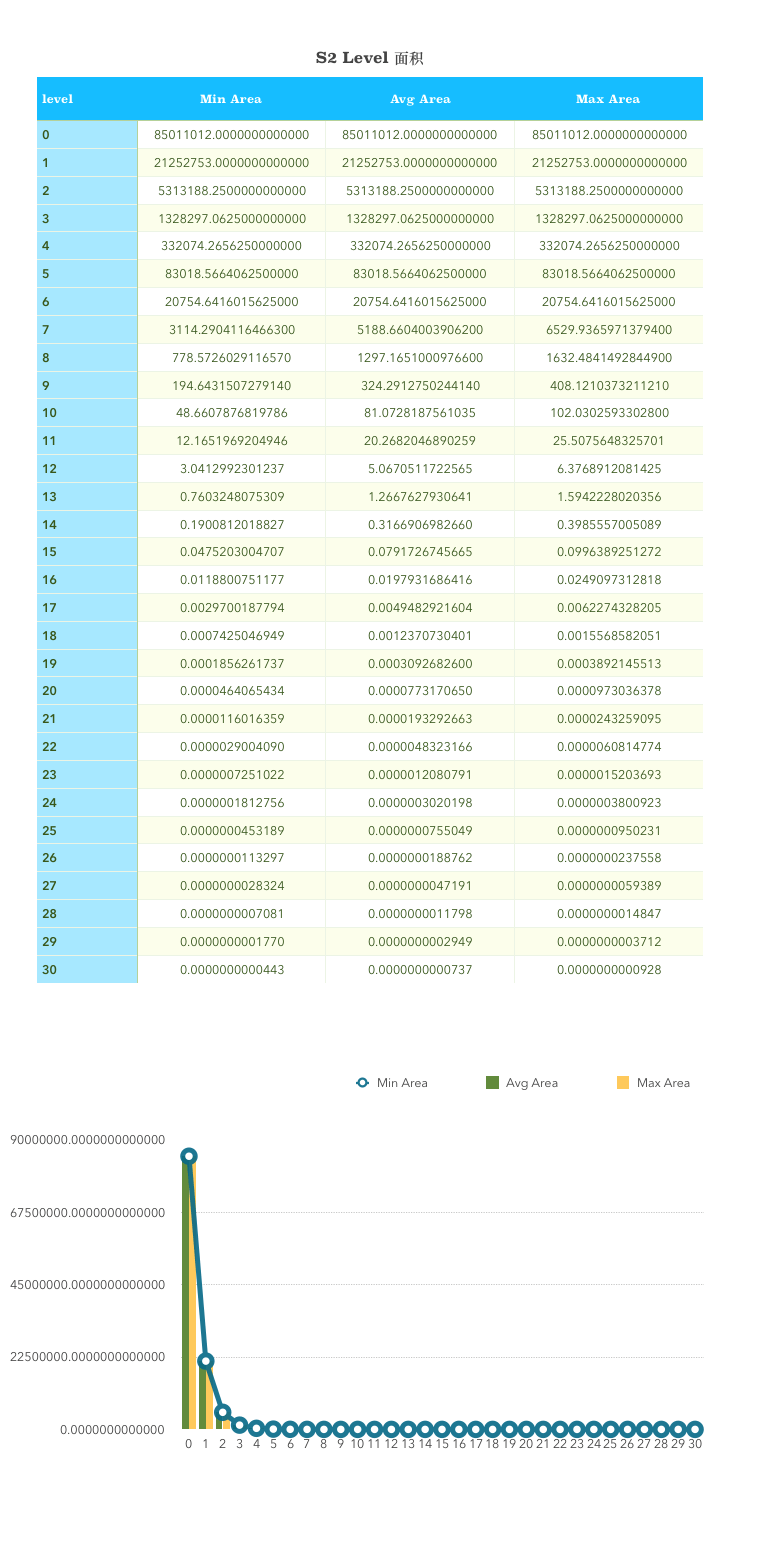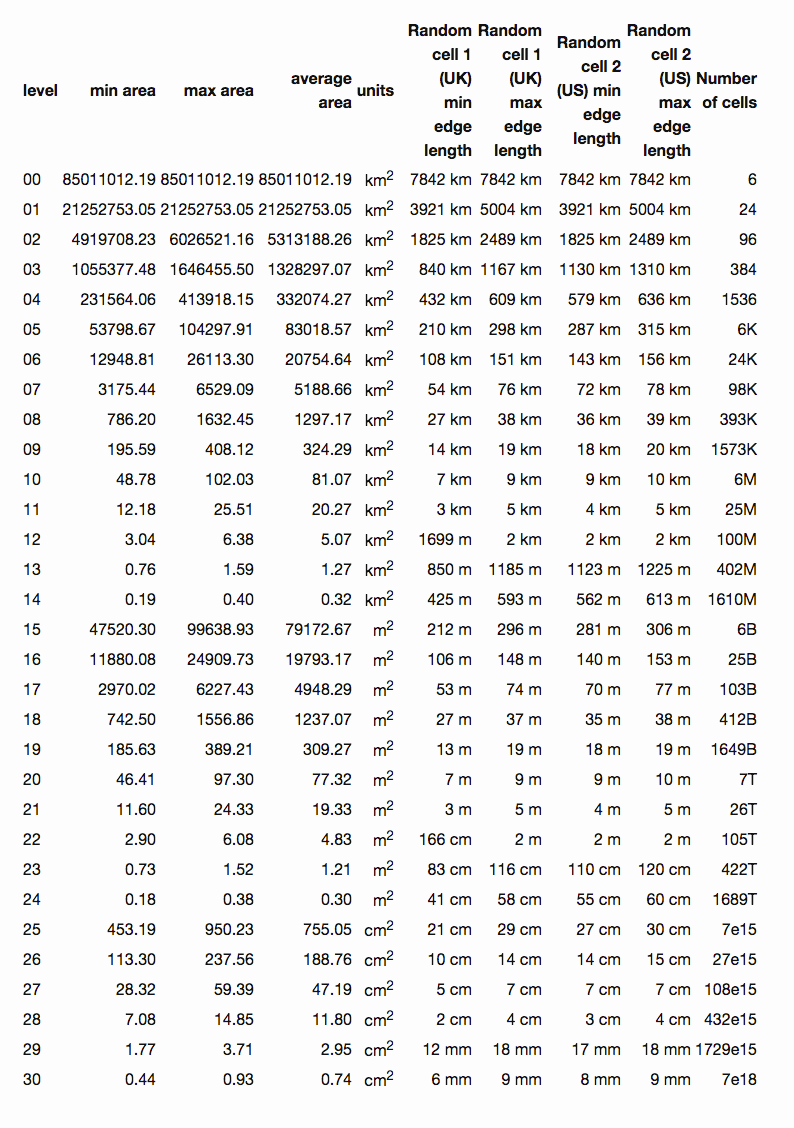level 0 就是正方体的六个面之一。地球表面积约等于510,100,000 km2。level 0 的面积就是地球表面积的六分之一。level 30 能表示的最小的面积0.48cm2，最大也就0.93cm2 。

### 7. S2 与 Geohash 对比

Geohash 有12级，从5000km 到 3.7cm。中间每一级的变化比较大。有时候可能选择上一级会大很多，选择下一级又会小一些。比如选择字符串长度为4，它对应的 cell 宽度是39.1km，需求可能是50km，那么选择字符串长度为5，对应的 cell 宽度就变成了156km，瞬间又大了3倍了。这种情况选择多长的 Geohash 字符串就比较难选。选择不好，每次判断可能就还需要取出周围的8个格子再次进行判断。Geohash 需要 12 bytes 存储。

S2 有30级，从 0.7cm² 到 85,000,000km² 。中间每一级的变化都比较平缓，接近于4次方的曲线。所以选择精度不会出现 Geohash 选择困难的问题。S2 的存储只需要一个 uint64 即可存下。

S2 库里面不仅仅有地理编码，还有其他很多几何计算相关的库。地理编码只是其中的一小部分。本文没有介绍到的 S2 的实现还有很多很多，各种向量计算，面积计算，多边形覆盖，距离问题，球面球体上的问题，它都有实现。

S2 还能解决多边形覆盖的问题。比如给定一个城市，求一个多边形刚刚好覆盖住这个城市。### 8. S2 Cell 举例

Go

`     latlng := s2.LatLngFromDegrees(31.232135, 121.41321700000003)     cellID := s2.CellIDFromLatLng(latlng)     cell := s2.CellFromCellID(cellID) //9279882742634381312      // cell.Level()     fmt.Println("latlng = ", latlng)     fmt.Println("cell level = ", cellID.Level())     fmt.Printf("cell = %d\n", cellID)     smallCell := s2.CellFromCellID(cellID.Parent(10))     fmt.Printf("smallCell level = %d\n", smallCell.Level())     fmt.Printf("smallCell id = %b\n", smallCell.ID())     fmt.Printf("smallCell ApproxArea = %v\n", smallCell.ApproxArea())     fmt.Printf("smallCell AverageArea = %v\n", smallCell.AverageArea())     fmt.Printf("smallCell ExactArea = %v\n", smallCell.ExactArea())`

Go

` latlng =  [31.2321350, 121.4132170] cell level =  30 cell = 3869277663051577529  ****Parent **** 10000000000000000000000000000000000000000 smallCell level = 10 smallCell id = 11010110110010011011110000000000000000000000000000000000000000 smallCell ApproxArea = 1.9611002454714756e-06 smallCell AverageArea = 1.997370817559429e-06 smallCell ExactArea = 1.9611009480261058e-06`

Go

`     rect = s2.RectFromLatLng(s2.LatLngFromDegrees(48.99, 1.852))     rect = rect.AddPoint(s2.LatLngFromDegrees(48.68, 2.75))      rc := &s2.RegionCoverer{MaxLevel: 20, MaxCells: 10, MinLevel: 2}     r := s2.Region(rect.CapBound())     covering := rc.Covering(r)`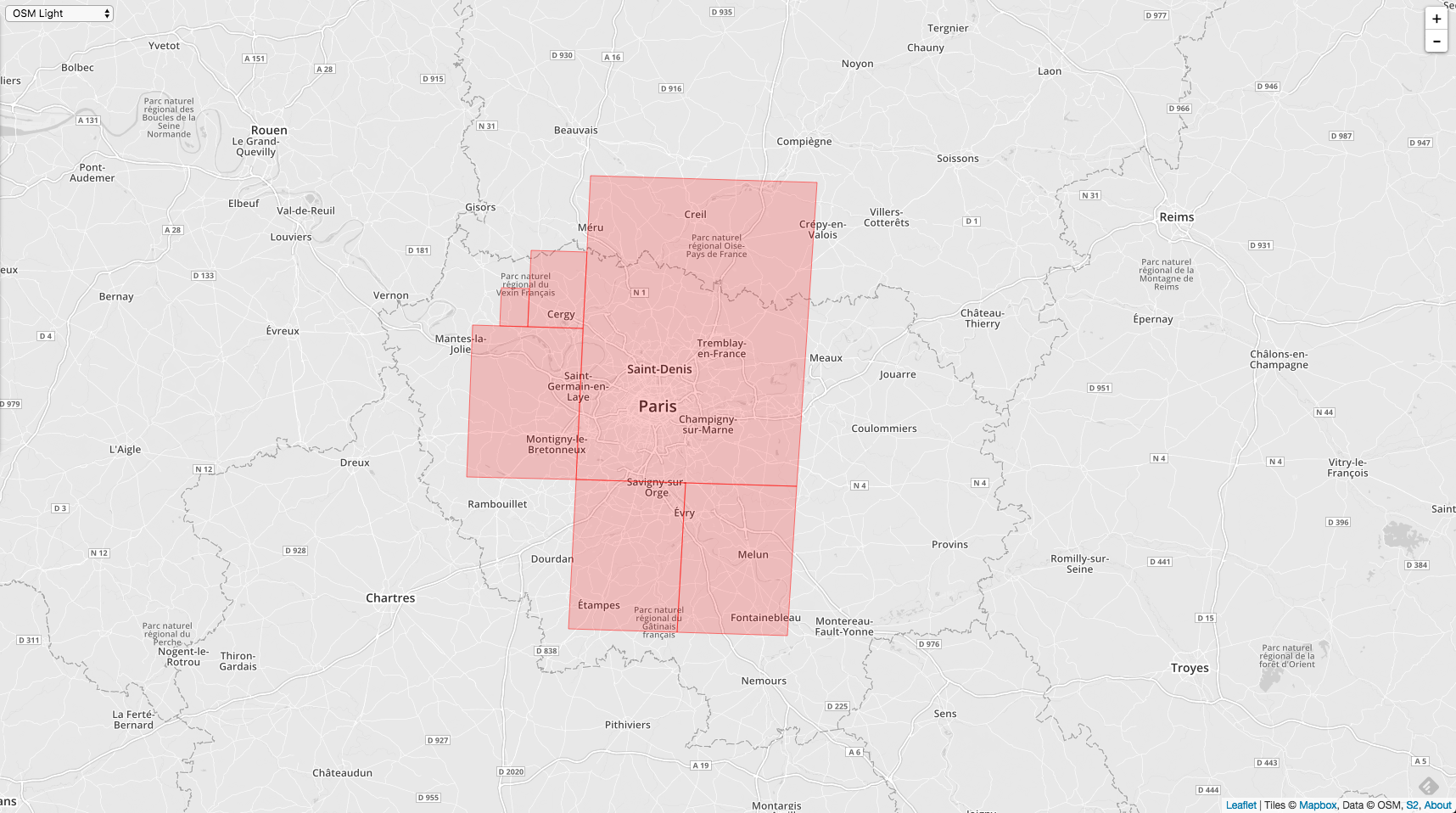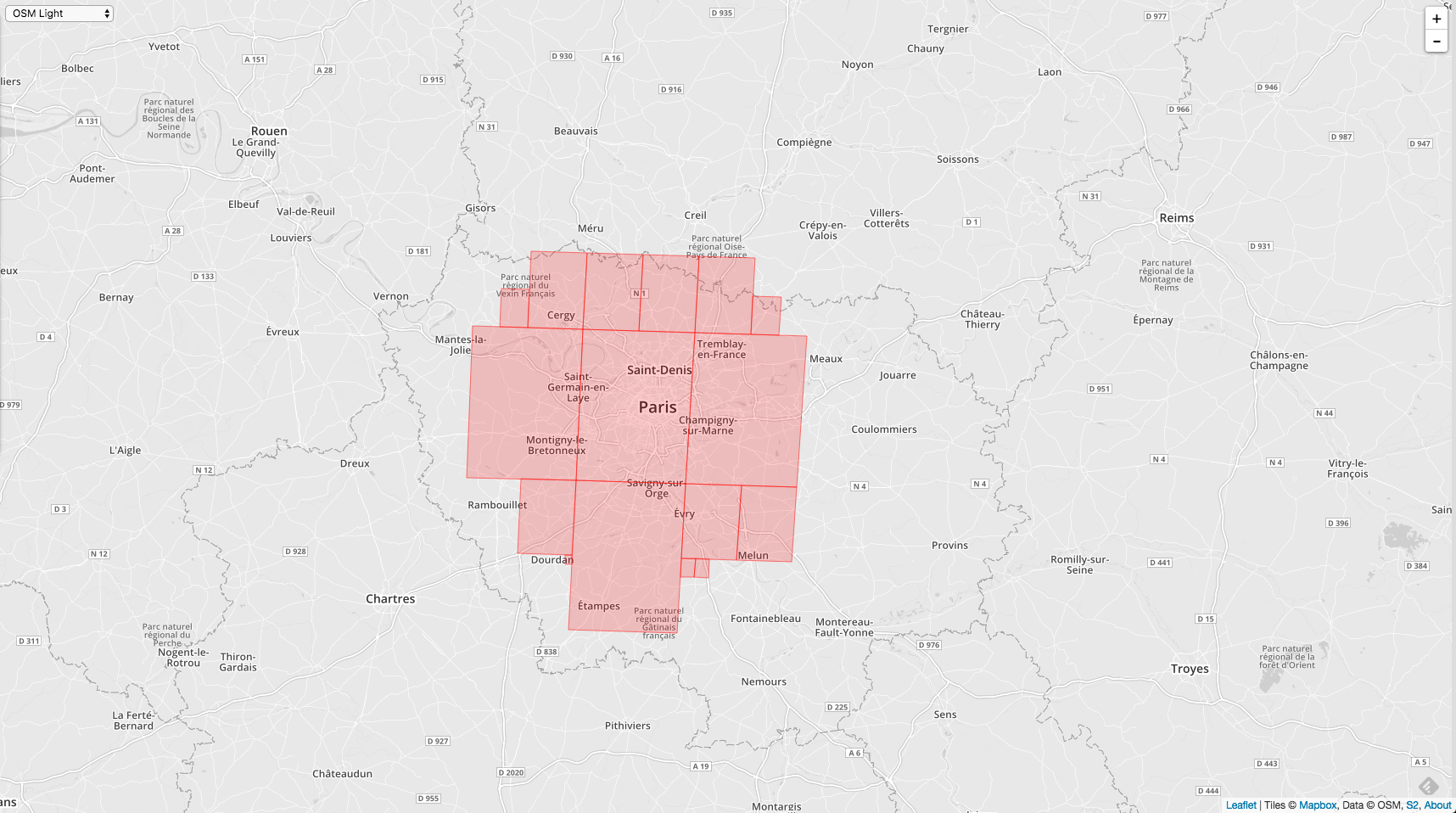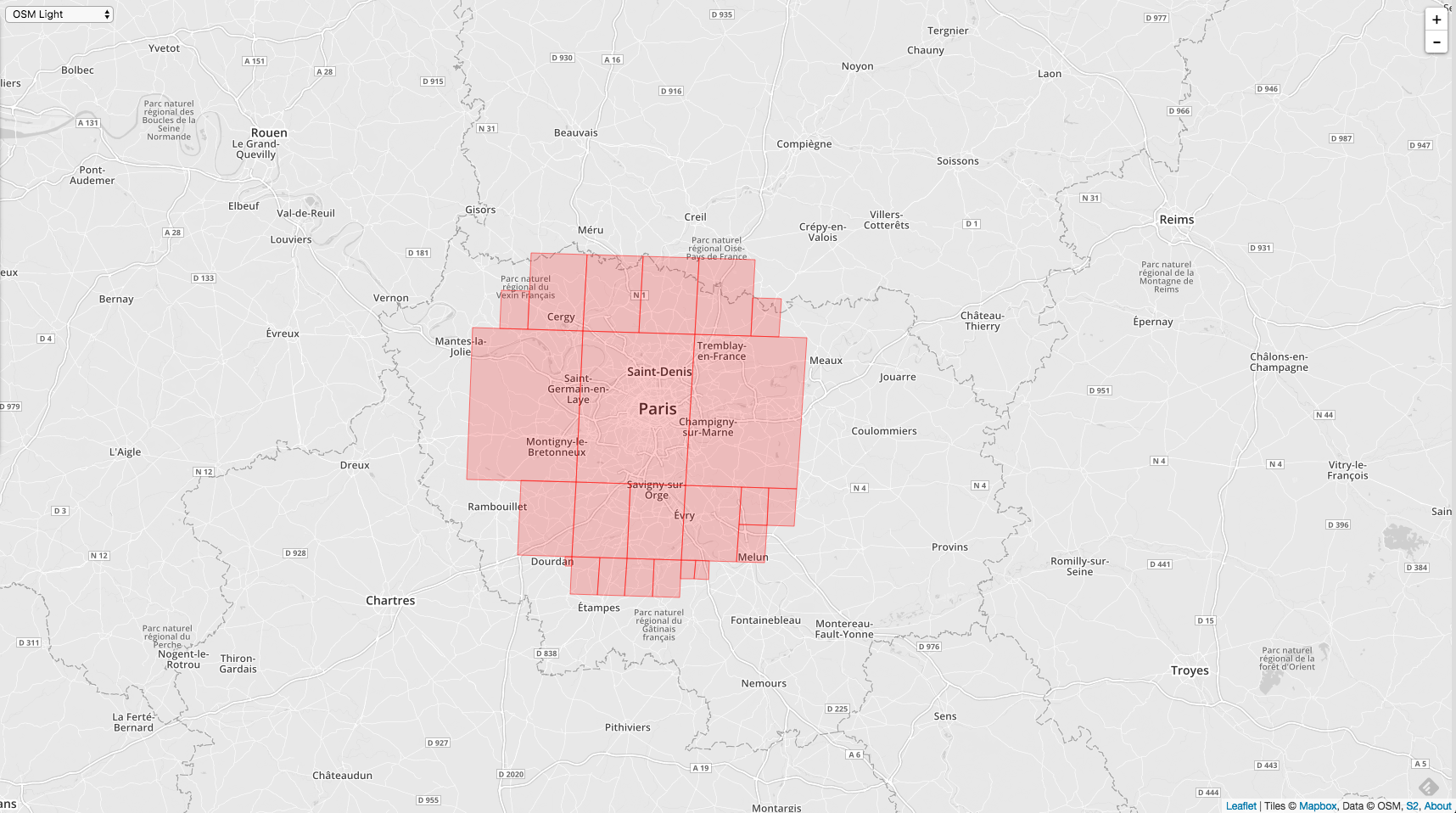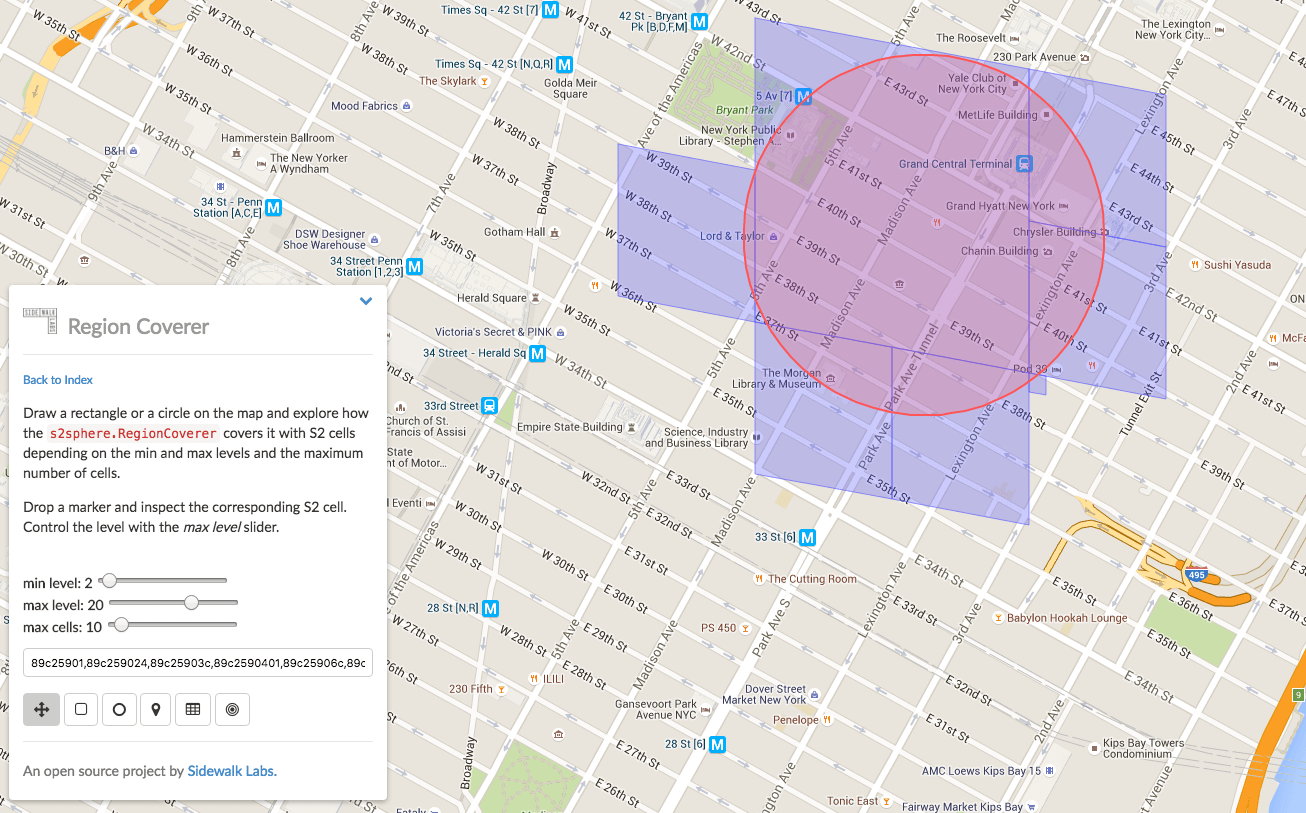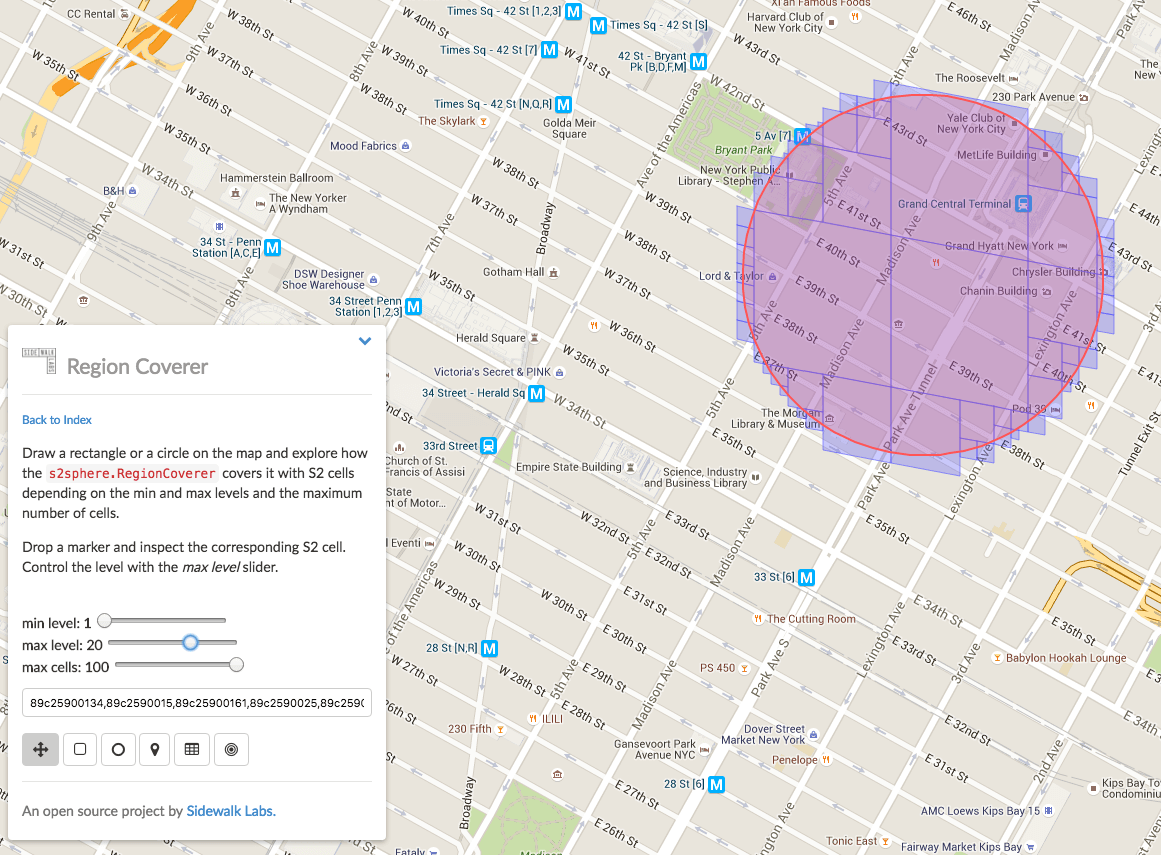Go

` func testLoop() {      ll1 := s2.LatLngFromDegrees(31.803269, 113.421145)     ll2 := s2.LatLngFromDegrees(31.461846, 113.695803)     ll3 := s2.LatLngFromDegrees(31.250756, 113.756228)     ll4 := s2.LatLngFromDegrees(30.902604, 113.997927)     ll5 := s2.LatLngFromDegrees(30.817726, 114.464846)     ll6 := s2.LatLngFromDegrees(30.850743, 114.76697)     ll7 := s2.LatLngFromDegrees(30.713884, 114.997683)     ll8 := s2.LatLngFromDegrees(30.430111, 115.42615)     ll9 := s2.LatLngFromDegrees(30.088491, 115.640384)     ll10 := s2.LatLngFromDegrees(29.907713, 115.656863)     ll11 := s2.LatLngFromDegrees(29.783833, 115.135012)     ll12 := s2.LatLngFromDegrees(29.712295, 114.728518)     ll13 := s2.LatLngFromDegrees(29.55473, 114.24512)     ll14 := s2.LatLngFromDegrees(29.530835, 113.717776)     ll15 := s2.LatLngFromDegrees(29.55473, 113.3772)     ll16 := s2.LatLngFromDegrees(29.678892, 112.998172)     ll17 := s2.LatLngFromDegrees(29.941039, 112.349978)     ll18 := s2.LatLngFromDegrees(30.040949, 112.025882)     ll19 := s2.LatLngFromDegrees(31.803269, 113.421145)      point1 := s2.PointFromLatLng(ll1)     point2 := s2.PointFromLatLng(ll2)     point3 := s2.PointFromLatLng(ll3)     point4 := s2.PointFromLatLng(ll4)     point5 := s2.PointFromLatLng(ll5)     point6 := s2.PointFromLatLng(ll6)     point7 := s2.PointFromLatLng(ll7)     point8 := s2.PointFromLatLng(ll8)     point9 := s2.PointFromLatLng(ll9)     point10 := s2.PointFromLatLng(ll10)     point11 := s2.PointFromLatLng(ll11)     point12 := s2.PointFromLatLng(ll12)     point13 := s2.PointFromLatLng(ll13)     point14 := s2.PointFromLatLng(ll14)     point15 := s2.PointFromLatLng(ll15)     point16 := s2.PointFromLatLng(ll16)     point17 := s2.PointFromLatLng(ll17)     point18 := s2.PointFromLatLng(ll18)     point19 := s2.PointFromLatLng(ll19)      points := []s2.Point{}     points = append(points, point19)     points = append(points, point18)     points = append(points, point17)     points = append(points, point16)     points = append(points, point15)     points = append(points, point14)     points = append(points, point13)     points = append(points, point12)     points = append(points, point11)     points = append(points, point10)     points = append(points, point9)     points = append(points, point8)     points = append(points, point7)     points = append(points, point6)     points = append(points, point5)     points = append(points, point4)     points = append(points, point3)     points = append(points, point2)     points = append(points, point1)      loop := s2.LoopFromPoints(points)      fmt.Println("----  loop search (gets too much) -----")     // fmt.Printf("Some loop status items: empty:%t   full:%t \n", loop.IsEmpty(), loop.IsFull())      // ref: https://github.com/golang/geo/issues/14#issuecomment-257064823     defaultCoverer := &s2.RegionCoverer{MaxLevel: 20, MaxCells: 1000, MinLevel: 1}     // rg := s2.Region(loop.CapBound())     // cvr := defaultCoverer.Covering(rg)     cvr := defaultCoverer.Covering(loop)      // fmt.Println(poly.CapBound())     for _, c3 := range cvr {         fmt.Printf("%d,\n", c3)     } }`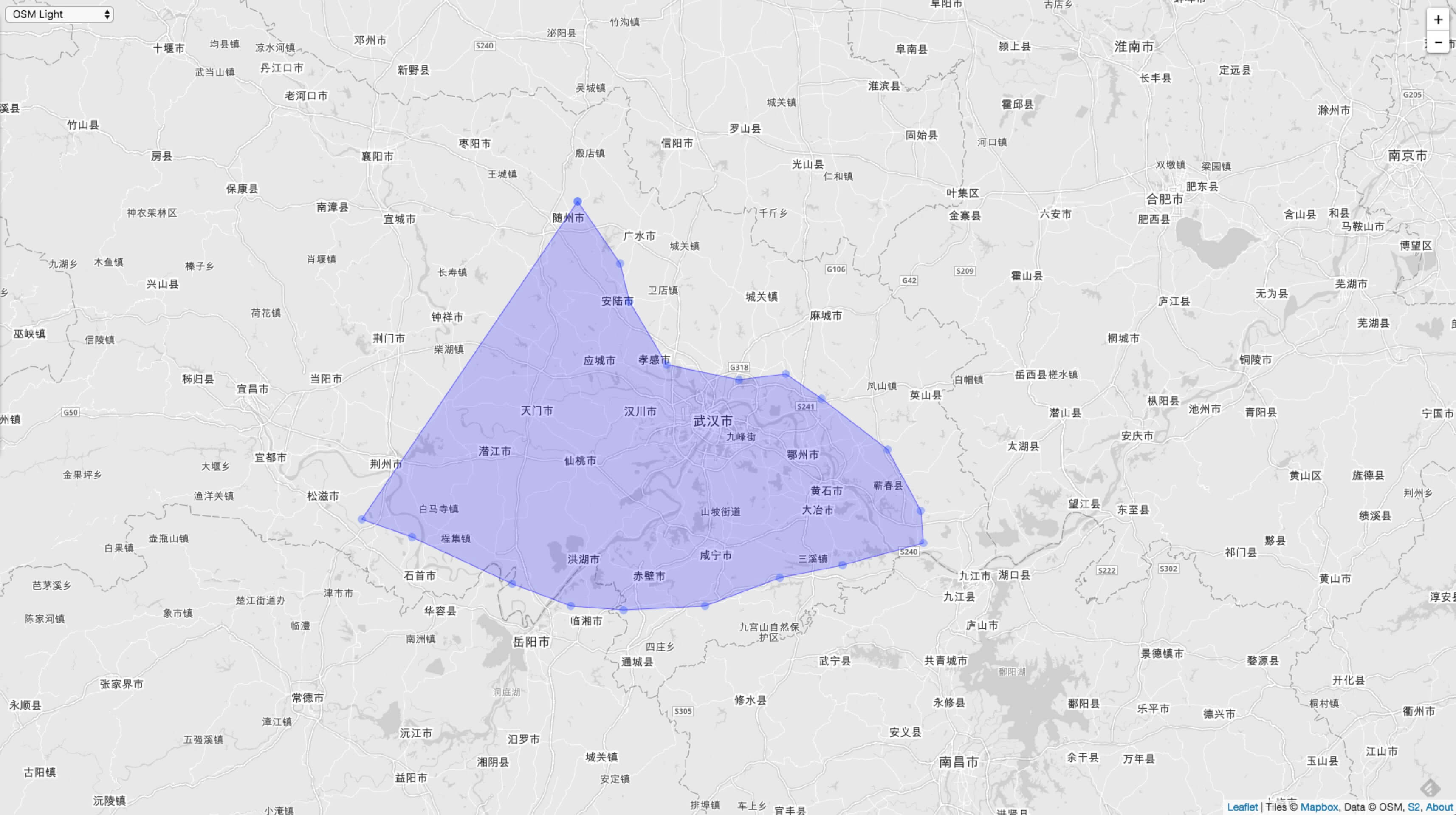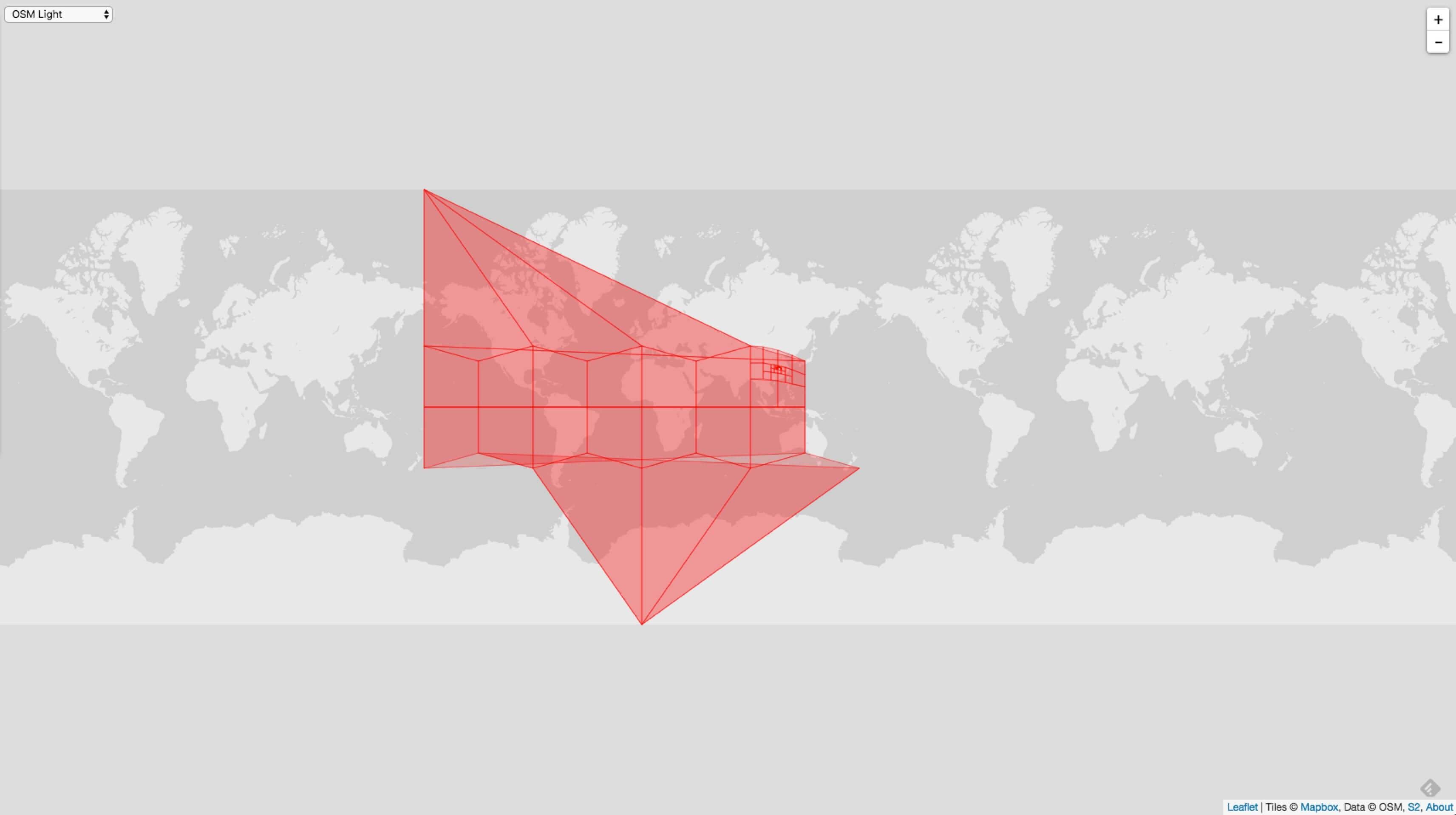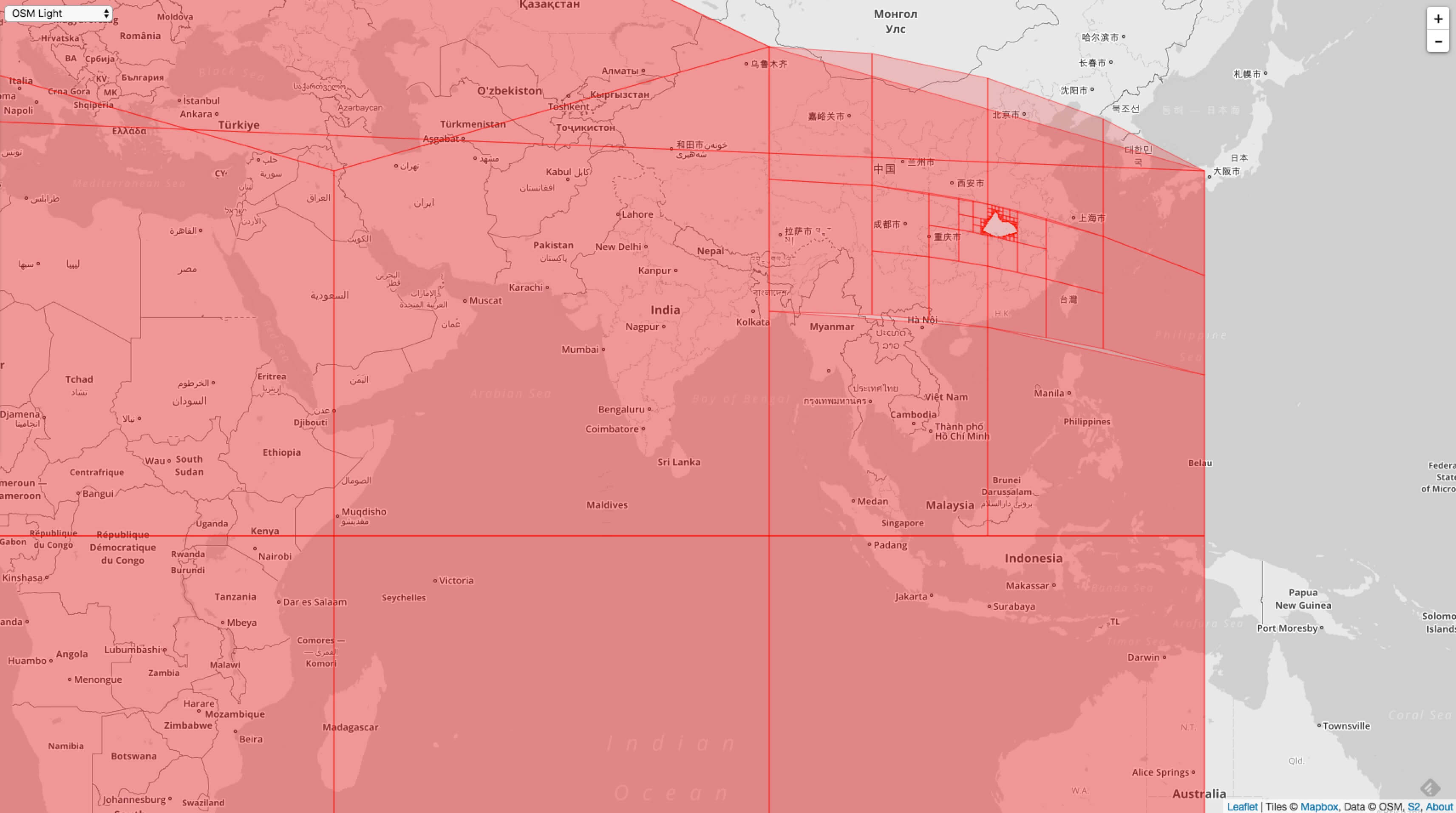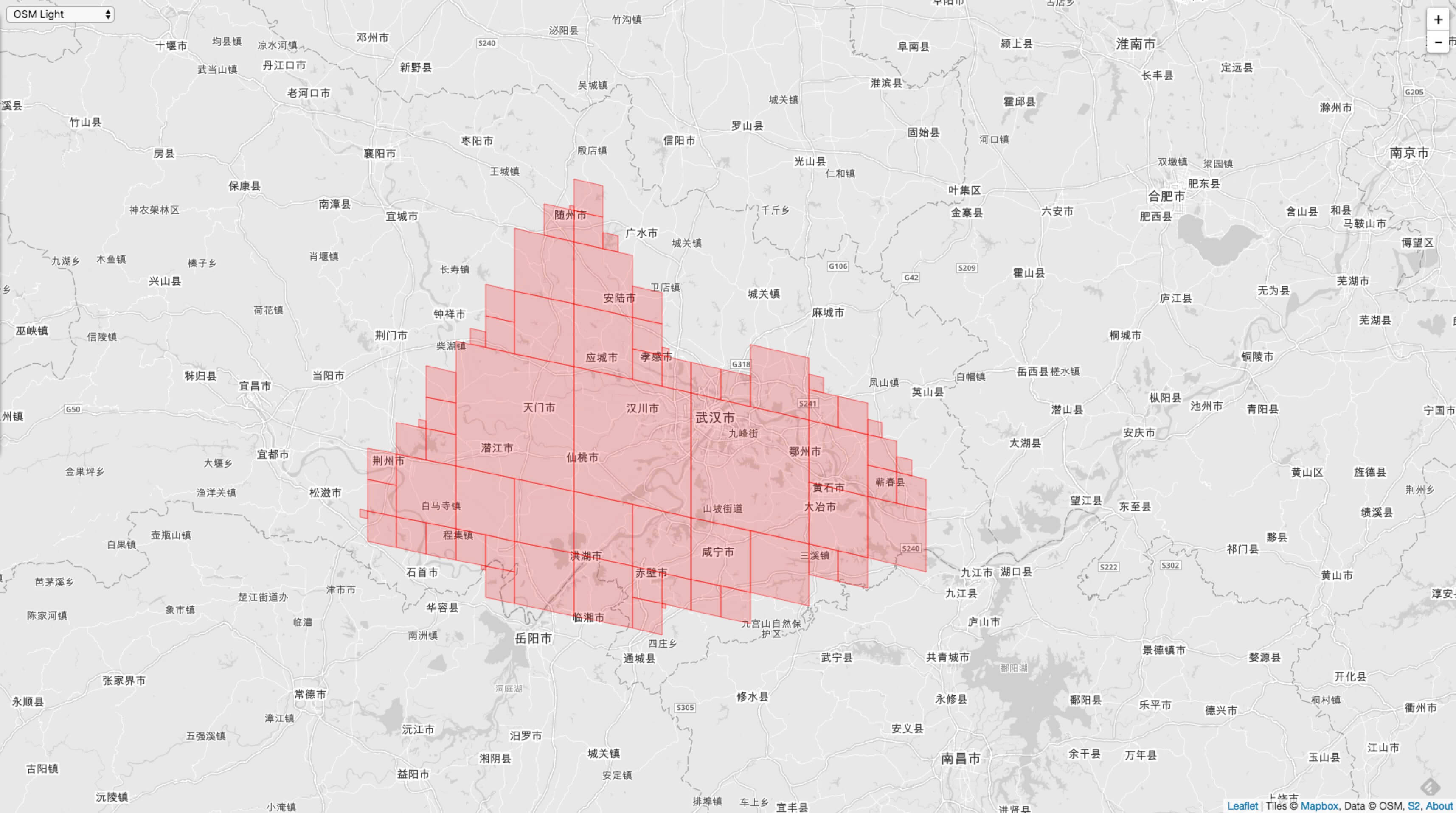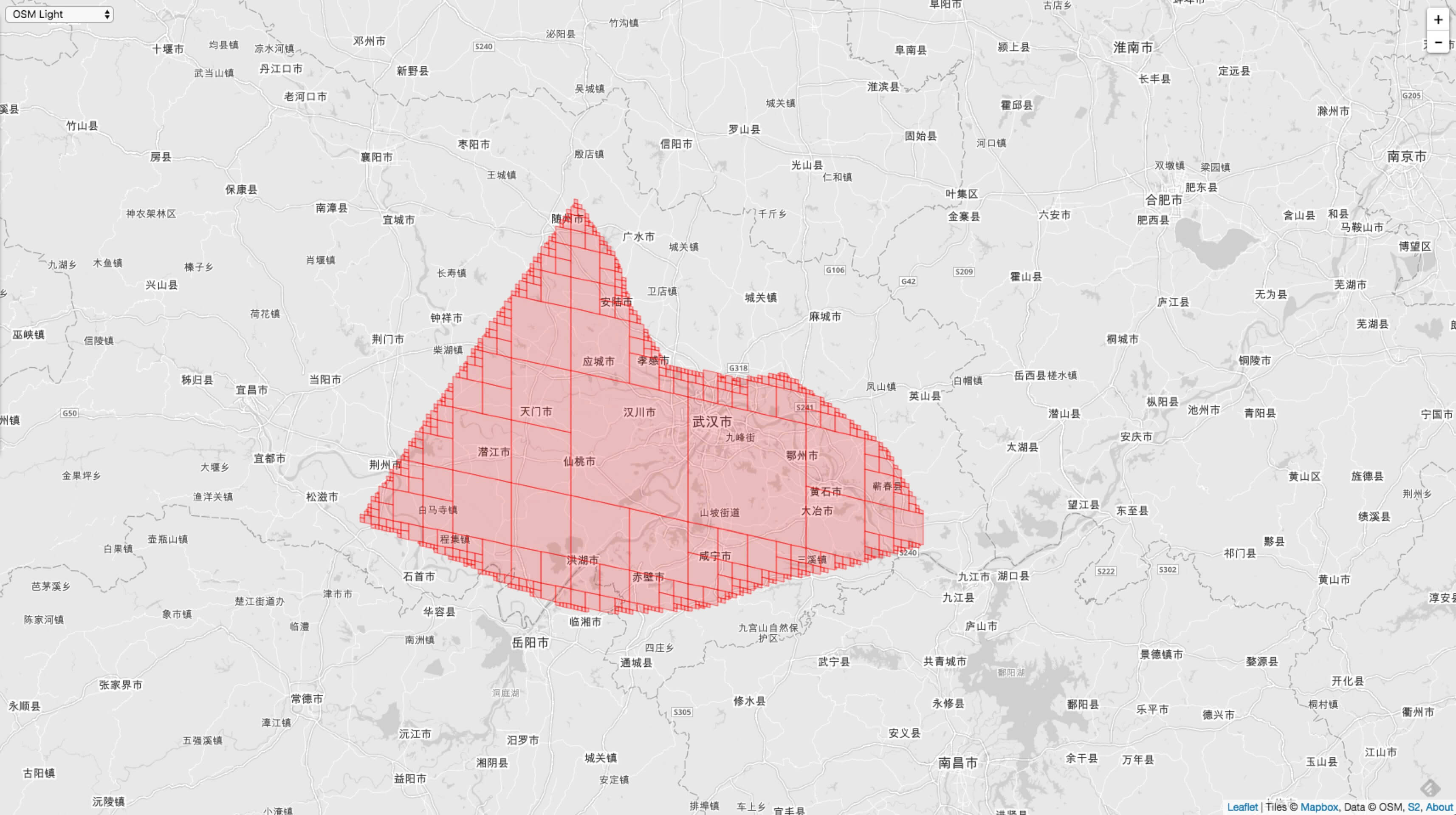### 9. S2 的应用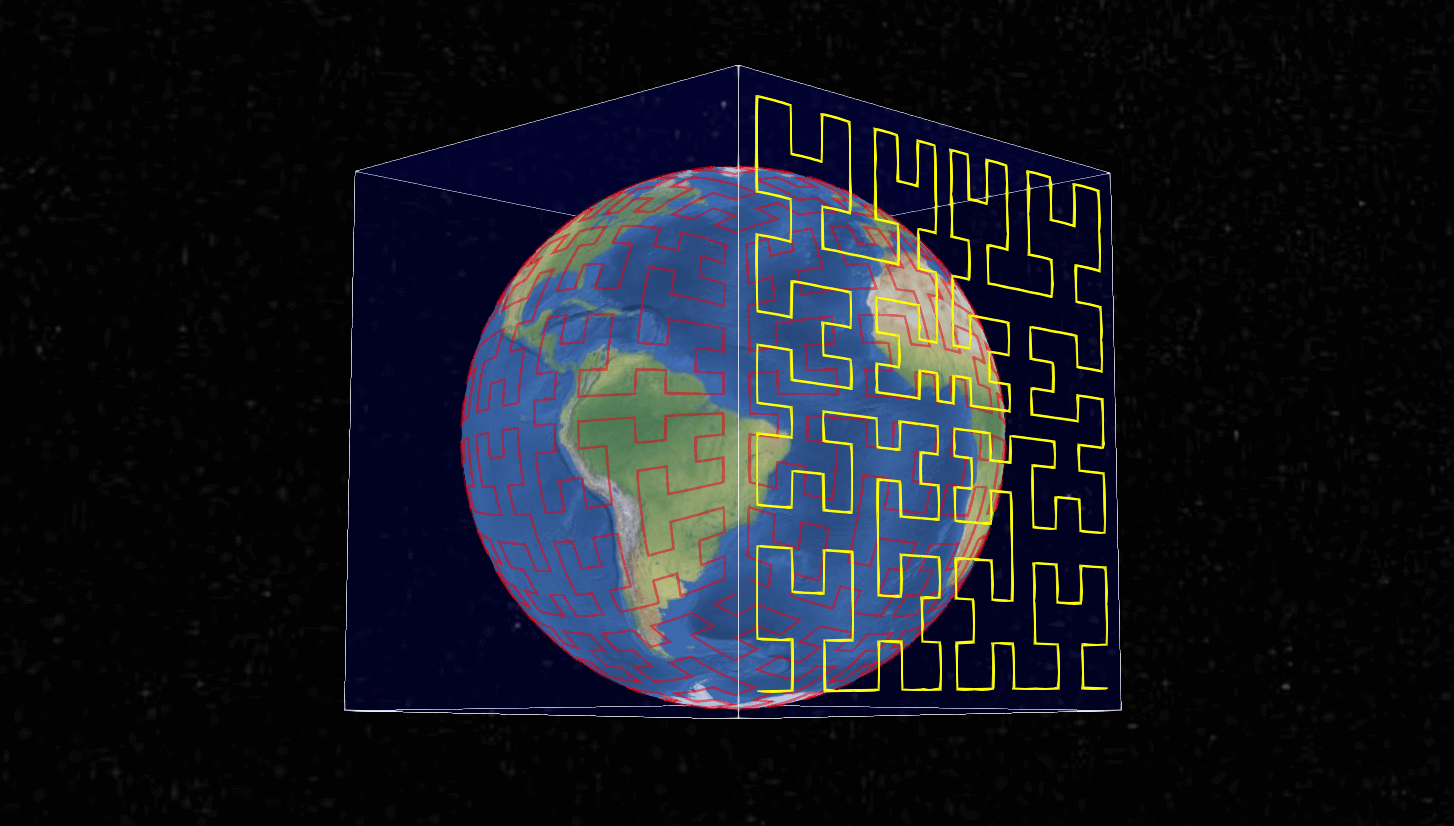S2 主要能用在以下 8 个地方：

1. 涉及到角度，间隔，纬度经度点，单位矢量等的表示，以及对这些类型的各种操作。
2. 单位球体上的几何形状，如球冠（“圆盘”），纬度 - 经度矩形，折线和多边形。
3. 支持点，折线和多边形的任意集合的强大的构造操作（例如联合）和布尔谓词（例如，包含）。
4. 对点，折线和多边形的集合进行快速的内存索引。
5. 针对测量距离和查找附近物体的算法。
6. 用于捕捉和简化几何的稳健算法（该算法具有精度和拓扑保证）。
7. 用于测试几何对象之间关系的有效且精确的数学谓词的集合。
8. 支持空间索引，包括将区域近似为离散“S2单元”的集合。此功能可以轻松构建大型分布式空间索引。

S2 目前应用比较多，用在和地图相关业务上更多。Google Map 就直接大量使用了 S2 ，速度有多快读者可以自己体验体验。Uber 在搜寻最近的出租车也是用的 S2 算法进行计算的。场景的例子就是本篇文章引语里面提到的场景。滴滴应该也有相关的应用，也许有更加优秀的解法。现在很火的共享单车也会用到这些空间索引算法。

1. 平面几何问题（有许多精细的现有平面几何图库可供选择）。
2. 转换常见的 to/from GIS格式（要阅读这种格式，请使用OGR等外部库）。

## 五. 最后GitHub Repo：Halfrost-Field

Follow: halfrost · GitHub